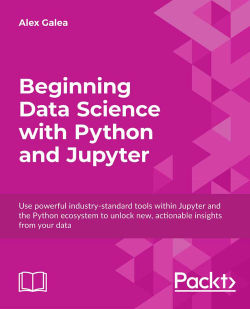### Beginning Data Science with Python and Jupyter4.5 (6 reviews total)
By Alex Galea
• FREE Subscribe Access now
• $14.99 Print + eBook •$11.99 eBook
• Constantly updated with 100+ new titles each month
• Breadth and depth in over 1,000+ technologies

Get to grips with the skills you need for entry-level data science in this hands-on Python and Jupyter course. You'll learn about some of the most commonly used libraries that are part of the Anaconda distribution, and then explore machine learning models with real datasets to give you the skills and exposure you need for the real world. We'll finish up by showing you how easy it can be to scrape and gather your own data from the open web, so that you can apply your new skills in an actionable context.

Publication date:
June 2018
Publisher
Packt
Pages
194
ISBN
9781789532029

## ChapterÂ 1.Â Jupyter Fundamentals

Jupyter Notebooks are one of the most important tools for data scientists using Python. This is because they're an ideal environment for developing reproducible data analysis pipelines. Data can be loaded, transformed, and modeled all inside a single Notebook, where it's quick and easy to test out code and explore ideas along the way. Furthermore, all of this can be documented "inline" using formatted text, so you can make notes for yourself or even produce a structured report.

Other comparable platforms - for example, RStudio or Spyder - present the user with multiple windows, which promote arduous tasks such as copy and pasting code around and rerunning code that has already been executed. These tools also tend to involve Read Eval Prompt Loops (REPLs) where code is run in a terminal session that has saved memory. This type of development environment is bad for reproducibility and not ideal for development either. Jupyter Notebooks solve all these issues by giving the user a single window where code snippets are executed and outputs are displayed inline. This lets users develop code efficiently and allows them to look back at previous work for reference, or even to make alterations.

We'll start the lesson by explaining exactly what Jupyter Notebooks are and continue to discuss why they are so popular among data scientists. Then, we'll open a Notebook together and go through some exercises to learn how the platform is used. Finally, we'll dive into our first analysis and perform an exploratory analysis in Basic Functionality and Features.

## Lesson Objectives

In this lesson, you will:

• Learn what a Jupyter Notebook is and why it's useful for data analysis

• Use Jupyter Notebook features

• Study Python data science libraries

• Perform simple exploratory data analysis

### Note

All code from this book are available as lesson-specific IPython notebooks in the code bundle. All color plots from this book are also available in the code bundle.

## Basic Functionality and Features

In this section, we first demonstrate the usefulness of Jupyter Notebooks with examples and through discussion. Then, in order to cover the fundamentals of Jupyter Notebooks for beginners, we'll see the basic usage of them in terms of launching and interacting with the platform. For those who have used Jupyter Notebooks before, this will be mostly a review; however, you will certainly see new things in this topic as well.

### Subtopic A: What is a Jupyter Notebook and Why is it Useful?

Jupyter Notebooks are locally run web applications which contain live code, equations, figures, interactive apps, and Markdown text. The standard language is Python, and that's what we'll be using for this book; however, note that a variety of alternatives are supported. This includes the other dominant data science language, R: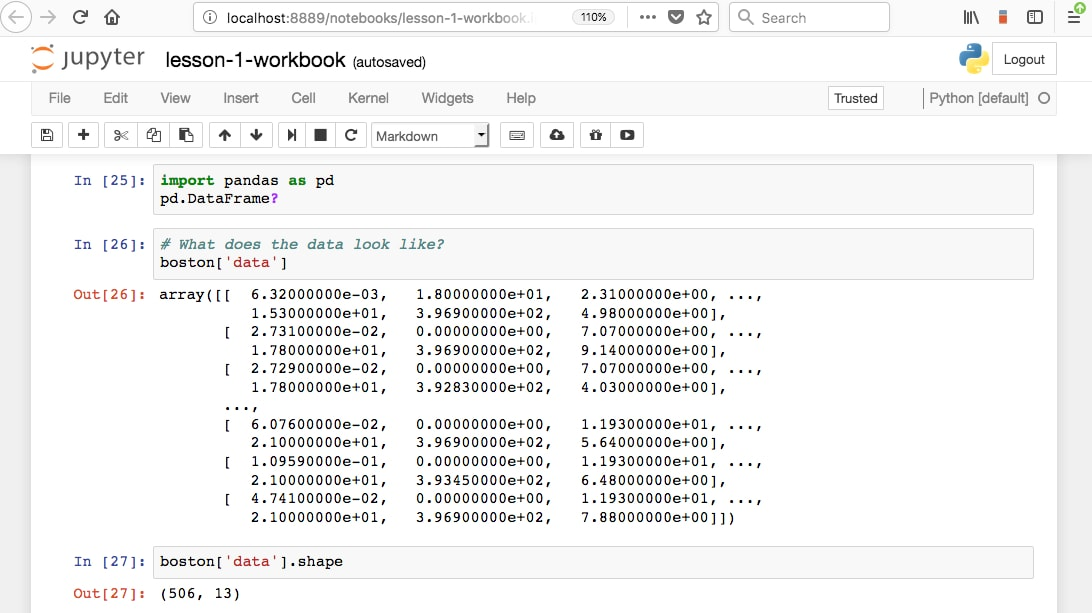Those familiar with R will know about R Markdown. Markdown documents allow for Markdown-formatted text to be combined with executable code. Markdown is a simple language used for styling text on the web. For example, most GitHub repositories have a README.md Markdown file. This format is useful for basic text formatting. It's comparable to HTML but allows for much less customization. Commonly used symbols in Markdown include hashes (#) to make text into a heading, square and round brackets to insert hyperlinks, and stars to create italicized or bold text: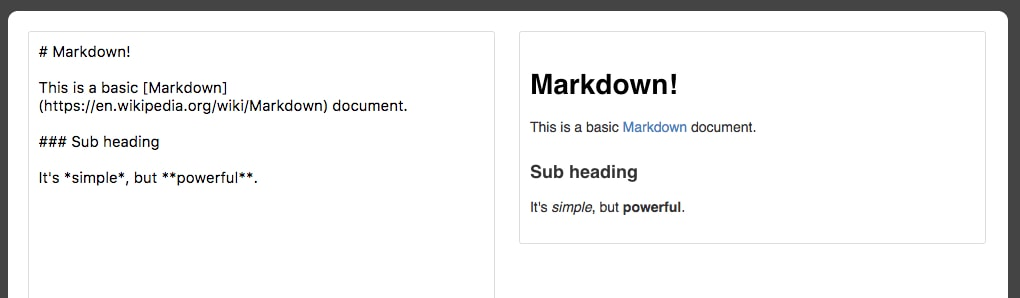Having seen the basics of Markdown, let's come back to R Markdown, where Markdown text can be written alongside executable code. Jupyter Notebooks offer the equivalent functionality for Python, although, as we'll see, they function quite differently than R Markdown documents. For example, R Markdown assumes you are writing Markdown unless otherwise specified, whereas Jupyter Notebooks assume you are inputting code. This makes it more appealing to use Jupyter Notebooks for rapid development and testing.

From a data science perspective, there are two primary types for a Jupyter Notebook depending on how they are used: lab-style and deliverable.

Lab-style Notebooks are meant to serve as the programming analog of research journals. These should contain all the work you've done to load, process, analyze, and model the data. The idea here is to document everything you've done for future reference, so it's usually not advisable to delete or alter previous lab-style Notebooks. It's also a good idea to accumulate multiple date-stamped versions of the Notebook as you progress through the analysis, in case you want to look back at previous states.

Deliverable Notebooks are intended to be presentable and should contain only select parts of the lab-style Notebooks. For example, this could be an interesting discovery to share with your colleagues, an in-depth report of your analysis for a manager, or a summary of the key findings for stakeholders.

In either case, an important concept is reproducibility. If you've been diligent in documenting your software versions, anyone receiving the reports will be able to rerun the Notebook and compute the same results as you did. In the scientific community, where reproducibility is becoming increasingly difficult, this is a breath of fresh air.

### Subtopic B: Navigating the Platform

Now, we are going to open up a Jupyter Notebook and start to learn the interface. Here, we will assume you have no prior knowledge of the platform and go over the basic usage.

#### Introducing Jupyter Notebooks

1. Navigate to the companion material directory in the terminal.

### Note

On Unix machines such as Mac or Linux, command-line navigation can be done using ls to display directory contents and cd to change directories.

On Windows machines, use dir to display directory contents and use cd to change directories instead. If, for example, you want to change the drive from C: to D:, you should execute d: to change drives.

2. Start a new local Notebook server here by typing the following into the terminal:

jupyter notebook

A new window or tab of your default browser will open the Notebook Dashboard to the working directory. Here, you will see a list of folders and files contained therein.

3. Click on a folder to navigate to that particular path and open a file by clicking on it. Although its main use is editing IPYNB Notebook files, Jupyter functions as a standard text editor as well.

4. Reopen the terminal window used to launch the app. We can see the NotebookApp being run on a local server. In particular, you should see a line like this:

[I 20:03:01.045 NotebookApp] The Jupyter Notebook is running at: http://localhost:8888/ ?token=e915bb06866f19ce462d959a9193a94c7c088e81765f9d8a

Going to that HTTP address will load the app in your browser window, as was done automatically when starting the app. Closing the window does not stop the app; this should be done from the terminal by typing Ctrl + C.

5. Close the app by typing Ctrl + C in the terminal. You may also have to confirm by entering y. Close the web browser window as well.

6. When loading the NotebookApp, there are various options available to you. In the terminal, see the list of available options by running the following:

jupyter notebook â€“-help
7. One such option is to specify a specific port. Open a NotebookApp at local port 9000 by running the following:

jupyter notebook --port 9000
8. The primary way to create a new Jupyter Notebook is from the Jupyter Dashboard. Click New in the upper-right corner and select a kernel from the drop-down menu (that is, select something in the Notebooks section):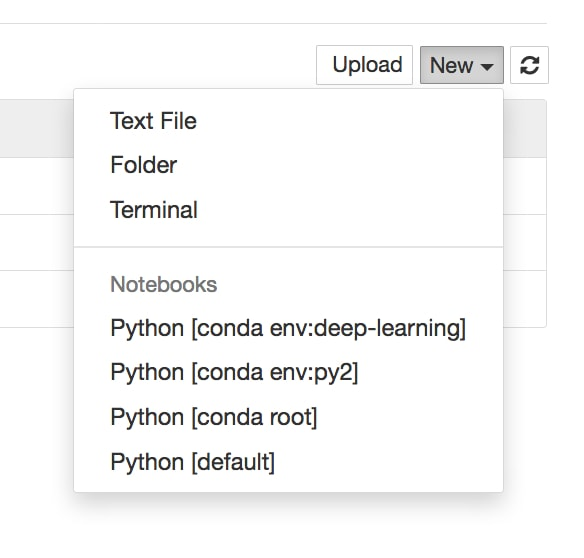Kernels provide programming language support for the Notebook. If you have installed Python with Anaconda, that version should be the default kernel. Conda virtual environments will also be available here.

### Note

Virtual environments are a great tool for managing multiple projects on the same machine. Each virtual environment may contain a different version of Python and external libraries. Python has built-in virtual environments; however, the Conda virtual environment integrates better with Jupyter Notebooks and boasts other nice features. The documentation is available at: https://conda.io/docs/user-guide/tasks/manage-environments.html.

9. With the newly created blank Notebook, click in the top cell and type print('hello world'), or any other code snippet that writes to the screen. Execute it by clicking in the cell and pressing Shift + Enter, or by selecting Run Cell in the Cell menu.

Any stdout or stderr output from the code will be displayed beneath as the cell runs. Furthermore, the string representation of the object written in the final line will be displayed as well. This is very handy, especially for displaying tables, but sometimes we don't want the final object to be displayed. In such cases, a semicolon (;) can be added to the end of the line to suppress the display.

New cells expect and run code input by default; however, they can be changed to render Markdown instead.

10. Click into an empty cell and change it to accept Markdown-formatted text. This can be done from the drop-down menu icon in the toolbar or by selecting Markdown from the Cell menu. Write some text in here (any text will do), making sure to utilize Markdown formatting symbols such as #.

11. Focus on the toolbar at the top of the Notebook: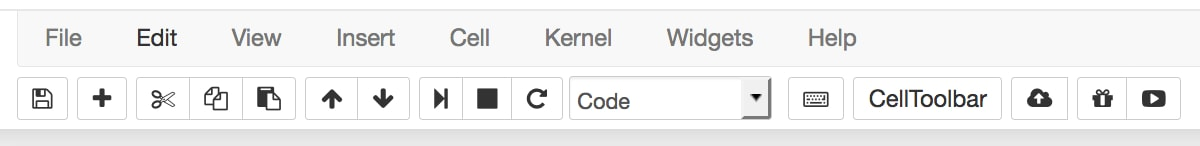There is a Play icon in the toolbar, which can be used to run cells. As we'll see later, however, it's handier to use the keyboard shortcut Shift + Enter to run cells. Right next to this is a Stop icon, which can be used to stop cells from running. This is useful, for example, if a cell is taking too long to run: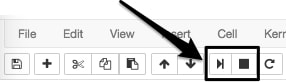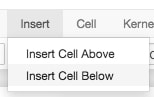Cells can be copied, pasted, and deleted using icons or by selecting options from the Edit menu: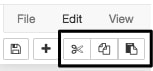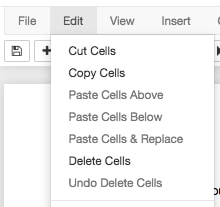Cells can also be moved up and down this way: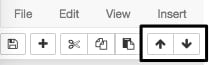There are useful options under the Cell menu to run a group of cells or the entire Notebook: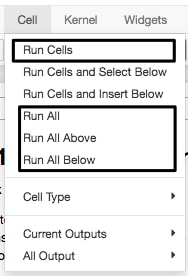12. Experiment with the toolbar options to move cells up and down, insert new cells, and delete cells.

An important thing to understand about these Notebooks is the shared memory between cells. It's quite simple: every cell existing on the sheet has access to the global set of variables. So, for example, a function defined in one cell could be called from any other, and the same applies to variables. As one would expect, anything within the scope of a function will not be a global variable and can only be accessed from within that specific function.

13. Open the Kernel menu to see the selections. The Kernel menu is useful for stopping script executions and restarting the Notebook if the kernel dies. Kernels can also be swapped here at any time, but it is unadvisable to use multiple kernels for a single Notebook due to reproducibility concerns.

14. Open the File menu to see the selections. The File menu contains options for downloading the Notebook in various formats. In particular, it's recommended to save an HTML version of your Notebook, where the content is rendered statically and can be opened and viewed "as you would expect" in web browsers.

The Notebook name will be displayed in the upper-left corner. New Notebooks will automatically be named Untitled.

15. Change the name of your IPYNB Notebook file by clicking on the current name in the upper-left corner and typing the new name. Then, save the file.

16. Close the current tab in your web browser (exiting the Notebook) and go to the Jupyter Dashboard tab, which should still be open. (If it's not open, then reload it by copy and pasting the HTTP link from the terminal.)

Since we didn't shut down the Notebook, we just saved and exited, it will have a green book symbol next to its name in the Files section of the Jupyter Dashboard and will be listed as Running on the right side next to the last modified date. Notebooks can be shut down from here.

17. Quit the Notebook you have been working on by selecting it (checkbox to the left of the name) and clicking the orange Shutdown button: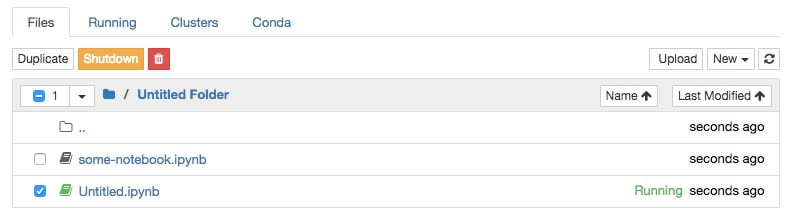### Note

If you plan to spend a lot of time working with Jupyter Notebooks, it's worthwhile to learn the keyboard shortcuts. This will speed up your workflow considerably. Particularly useful commands to learn are the shortcuts for manually adding new cells and converting cells from code to Markdown formatting. Click on Keyboard Shortcuts from the Help menu to see how.

### Subtopic C: Jupyter Features

Jupyter has many appealing features that make for efficient Python programming. These include an assortment of things, from methods for viewing docstrings to executing Bash commands. Let's explore some of these features together in this section.

### Note

The official IPython documentation can be found here: http://ipython.readthedocs.io/en/stable/. It has details on the features we will discuss here and others.

#### Explore some of Jupyter's most useful features

1. From the Jupyter Dashboard, navigate to the lesson-1 directory and open the lesson-1-workbook.ipynb file by selecting it. The standard file extension for Jupyter Notebooks is .ipynb, which was introduced back when they were called IPython Notebooks.

2. Scroll down to Subtopic C: Jupyter Features in the Jupyter Notebook. We start by reviewing the basic keyboard shortcuts. These are especially helpful to avoid having to use the mouse so often, which will greatly speed up the workflow. Here are the most useful keyboard shortcuts. Learning to use these will greatly improve your experience with Jupyter Notebooks as well as your own efficiency:

• Shift + Enter is used to run a cell

• The Esc key is used to leave a cell

• The M key is used to change a cell to Markdown (after pressing Esc)

• The Y key is used to change a cell to code (after pressing Esc)

• Arrow keys move cells (after pressing Esc)

• The Enter key is used to enter a cell

Moving on from shortcuts, the help option is useful for beginners and experienced coders alike. It can help provide guidance at each uncertain step.

Users can get help by adding a question mark to the end of any object and running the cell. Jupyter finds the docstring for that object and returns it in a pop-out window at the bottom of the app.

3. Run the Getting Help section cells and check out how Jupyter displays the docstrings at the bottom of the Notebook. Add a cell in this section and get help on the object of your choice: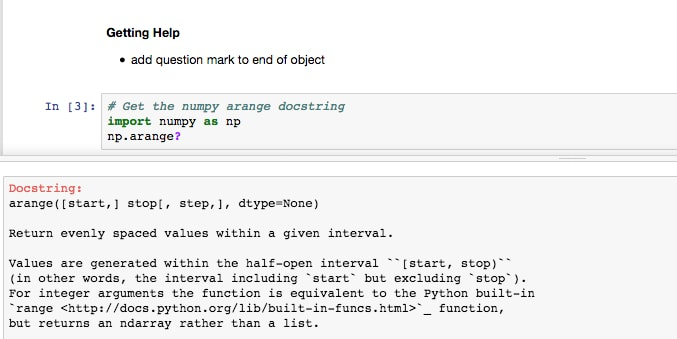Tab completion can be used to do the following:

• List available modules when importing external libraries

• List available modules of imported external libraries

• Function and variable completion

This can be especially useful when you need to know the available input arguments for a module, when exploring a new library, to discover new modules, or simply to speed up workflow. They will save time writing out variable names or functions and reduce bugs from typos. The tab completion works so well that you may have difficulty coding Python in other editors after today!

4. Click into an empty code cell in the Tab Completion section and try using tab completion in the ways suggested immediately above. For example, the first suggestion can be done by typing import (including the space after) and then pressing the Tab key: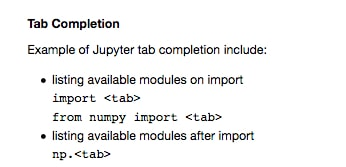5. Last but not least of the basic Jupyter Notebook features are magic commands. These consist of one or two percent signs followed by the command. Magics starting with %% will apply to the entire cell, and magics starting with % will only apply to that line. This will make sense when seen in an example.

Scroll to the Jupyter Magic Functions section and run the cells containing %lsmagic and %matplotlib inline: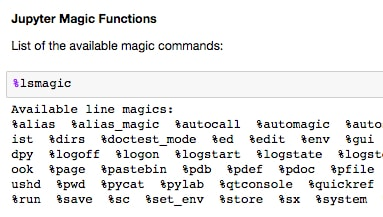%lsmagic lists the available options. We will discuss and show examples of some of the most useful ones. The most common magic command you will probably see is %matplotlib inline, which allows matplotlib figures to be displayed in the Notebook without having to explicitly use plt.show().

The timing functions are very handy and come in two varieties: a standard timer (%time or %%time) and a timer that measures the average runtime of many iterations (%timeit and %%timeit).

6. Run the cells in the Timers section. Note the difference between using one and two percent signs.

Even by using a Python kernel (as you are currently doing), other languages can be invoked using magic commands. The built-in options include JavaScript, R, Pearl, Ruby, and Bash. Bash is particularly useful, as you can use Unix commands to find out where you are currently (pwd), what's in the directory (ls), make new folders (mkdir), and write file contents (cat / head / tail).

7. Run the first cell in the Using bash in the notebook section. This cell writes some text to a file in the working directory, prints the directory contents, prints an empty line, and then writes back the contents of the newly created file before removing it: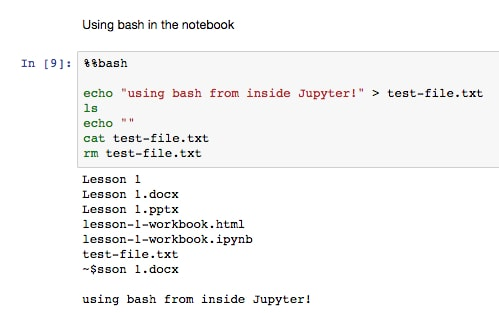8. Run the following cells containing only ls and pwd. Note how we did not have to explicitly use the Bash magic command for these to work.

There are plenty of external magic commands that can be installed. A popular one is ipython-sql, which allows for SQL code to be executed in cells.

9. If you've not already done so, install ipython-sql now. Open a new terminal window and execute the following code:

pip install ipython-sql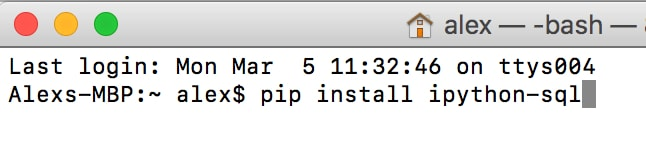10. Run the %load_ext sql cell to load the external command into the Notebook: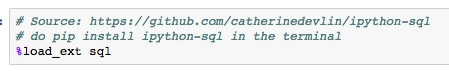This allows for connections to remote databases so that queries can be executed (and thereby documented) right inside the Notebook.

11. Run the cell containing the SQL sample query: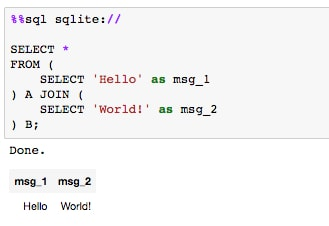Here, we first connect to the local sqlite source; however, this line could instead point to a specific database on a local or remote server. Then, we execute a simple SELECT to show how the cell has been converted to run SQL code instead of Python.

12. Moving on to other useful magic functions, we'll briefly discuss one that helps with documentation. The command is %version_information, but it does not come as standard with Jupyter. Like the SQL one we just saw, it can be installed from the command line with pip.

If not already done, install the version documentation tool now from the terminal using pip. Open up a new window and run the following code:

pip install version_information

Once installed, it can then be imported into any Notebook using %load_ext version_information. Finally, once loaded, it can be used to display the versions of each piece of software in the Notebook.

13. Run the cell that loads and calls the version_information command: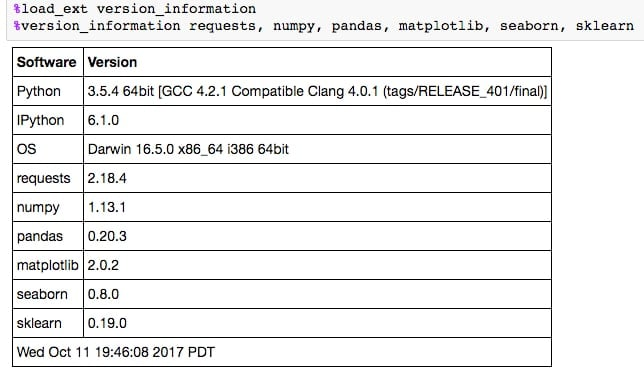#### Converting a Jupyter Notebook to a Python Script

You can convert a Jupyter Notebook to a Python script. This is equivalent to copying and pasting the contents of each code cell into a single .py file. The Markdown sections are also included as comments.

The conversion can be done from the NotebookApp or in the command line as follows:

jupyter nbconvert --to=python lesson-1-notebook.ipynb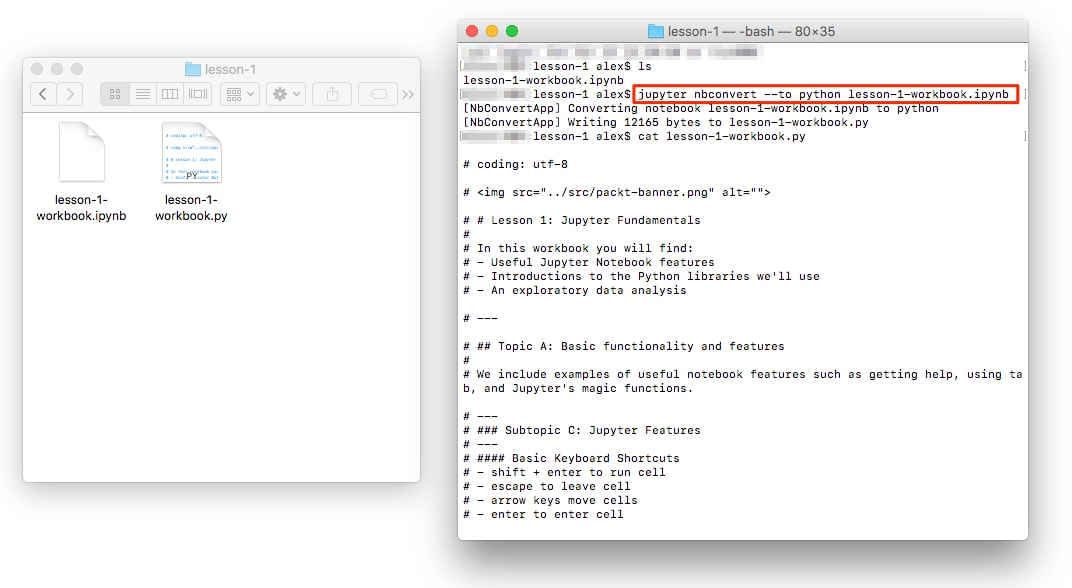This is useful, for example, when you want to determine the library requirements for a Notebook using a tool such as pipreqs. This tool determines the libraries used in a project and exports them into a requirements.txt file (and it can be installed by running pip install pipreqs).

The command is called from outside the folder containing your .py files. For example, if the .py files are inside a folder called lesson-1, you could do the following:

pipreqs lesson-1/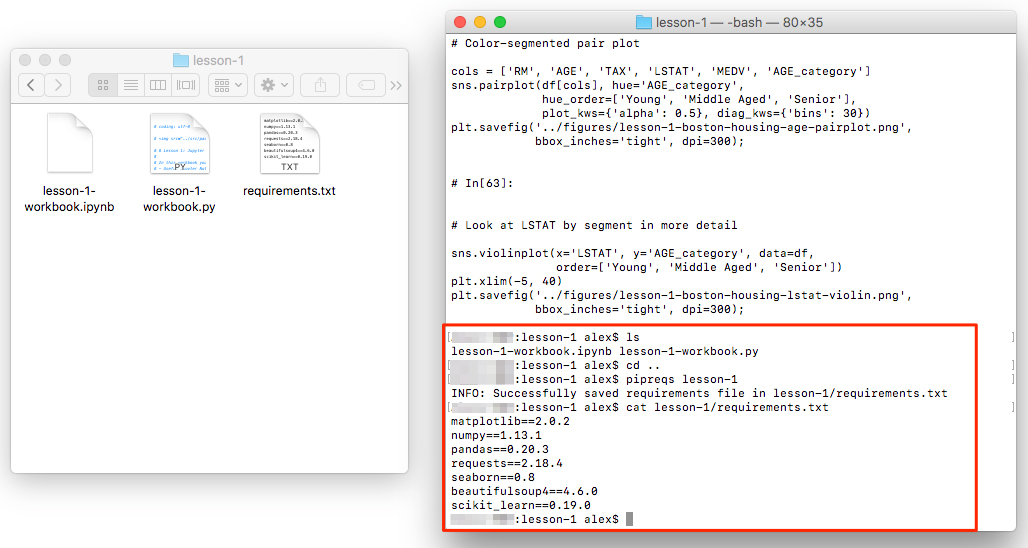The resulting requirements.txt file for lesson-1-workbook.ipynb looks like this:

cat lesson-1/requirements.txt
matplotlib==2.0.2
numpy==1.13.1
pandas==0.20.3
requests==2.18.4
seaborn==0.8
beautifulsoup4==4.6.0
scikit_learn==0.19.0

### Subtopic D: Python Libraries

Having now seen all the basics of Jupyter Notebooks, and even some more advanced features, we'll shift our attention to the Python libraries we'll be using in this book. Libraries, in general, extend the default set of Python functions. Examples of commonly used standard libraries are datetime, time, and os. These are called standard libraries because they come standard with every installation of Python.

For data science with Python, the most important libraries are external, which means they do not come standard with Python.

The external data science libraries we'll be using in this book are NumPy, Pandas, Seaborn, matplotlib, scikit-learn, Requests, and Bokeh. Let's briefly introduce each.

### Note

It's a good idea to import libraries using industry standards, for example, import numpy as np; this way, your code is more readable. Try to avoid doing things such as from numpy import *, as you may unwittingly overwrite functions. Furthermore, it's often nice to have modules linked to the library via a dot (.) for code readability.

• NumPy offers multi-dimensional data structures (arrays) on which operations can be performed far quicker than standard Python data structures (for example, lists). This is done in part by performing operations in the background using C. NumPy also offers various mathematical and data manipulation functions.

• Pandas is Python's answer to the R DataFrame. It stores data in 2D tabular structures where columns represent different variables and rows correspond to samples. Pandas provides many handy tools for data wrangling such as filling in NaN entries and computing statistical descriptions of the data. Working with Pandas DataFrames will be a big focus of this book.

• Matplotlib is a plotting tool inspired by the MATLAB platform. Those familiar with R can think of it as Python's version of ggplot. It's the most popular Python library for plotting figures and allows for a high level of customization.

• Seaborn works as an extension to matplotlib, where various plotting tools useful for data science are included. Generally speaking, this allows for analysis to be done much faster than if you were to create the same things manually with libraries such as matplotlib and scikit-learn.

• scikit-learn is the most commonly used machine learning library. It offers top-of-the-line algorithms and a very elegant API where models are instantiated and then fit with data. It also provides data processing modules and other tools useful for predictive analytics.

• Requests is the go-to library for making HTTP requests. It makes it straightforward to get HTML from web pages and interface with APIs. For parsing the HTML, many choose BeautifulSoup4, which we will also cover in this book.

• Bokeh is an interactive visualization library. It functions similar to matplotlib, but allows us to add hover, zoom, click, and use other interactive tools to our plots. It also allows us to render and play with the plots inside our Jupyter Notebook.

Having introduced these libraries, let's go back to our Notebook and load them, by running the import statements. This will lead us into our first analysis, where we finally start working with a dataset.

#### Import the external libraries and set up the plotting environment

1. Open up the lesson 1 Jupyter Notebook and scroll to the Subtopic D: Python Libraries section.

Just like for regular Python scripts, libraries can be imported into the Notebook at any time. It's best practice to put the majority of the packages you use at the top of the file. Sometimes it makes sense to load things midway through the Notebook and that is completely OK.

2. Run the cells to import the external libraries and set the plotting options: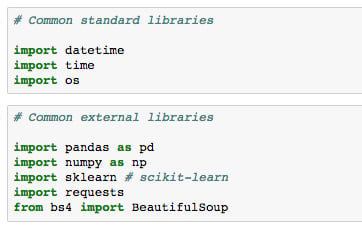For a nice Notebook setup, it's often useful to set various options along with the imports at the top. For example, the following can be run to change the figure appearance to something more aesthetically pleasing than the matplotlib and Seaborn defaults:

import matplotlib.pyplot as plt
%matplotlib inline
import seaborn as sns
# See here for more options: https://matplotlib.org/users/customizing.html
%config InlineBackend.figure_format='retina'
sns.set() # Revert to matplotlib defaults
plt.rcParams['figure.figsize'] = (9, 6)
sns.set_style("darkgrid")

So far in this book, we've gone over the basics of using Jupyter Notebooks for data science. We started by exploring the platform and finding our way around the interface. Then, we discussed the most useful features, which include tab completion and magic functions. Finally, we introduced the Python libraries we'll be using in this book.

The next section will be very interactive as we perform our first analysis together using the Jupyter Notebook.

## Our First Analysis - The Boston Housing Dataset

So far, this lesson has focused on the features and basic usage of Jupyter. Now, we'll put this into practice and do some data exploration and analysis.

The dataset we'll look at in this section is the so-called Boston housing dataset. It contains US census data concerning houses in various areas around the city of Boston. Each sample corresponds to a unique area and has about a dozen measures. We should think of samples as rows and measures as columns. The data was first published in 1978 and is quite small, containing only about 500 samples.

Now that we know something about the context of the dataset, let's decide on a rough plan for the exploration and analysis. If applicable, this plan would accommodate the relevant question(s) under study. In this case, the goal is not to answer a question but to instead show Jupyter in action and illustrate some basic data analysis methods.

Our general approach to this analysis will be to do the following:

• Load the data into Jupyter using a Pandas DataFrame

• Quantitatively understand the features

• Look for patterns and generate questions

• Answer the questions to the problems

### Subtopic A: Loading the Data into Jupyter Using a Pandas DataFrame

Oftentimes, data is stored in tables, which means it can be saved as a comma-separated variable (CSV) file. This format, and many others, can be read into Python as a DataFrame object, using the Pandas library. Other common formats include tab-separated variable (TSV), SQL tables, and JSON data structures. Indeed, Pandas has support for all of these. In this example, however, we are not going to load the data this way because the dataset is available directly through scikit-learn.

### Note

An important part after loading data for analysis is ensuring that it's clean. For example, we would generally need to deal with missing data and ensure that all columns have the correct datatypes. The dataset we use in this section has already been cleaned, so we will not need to worry about this. However, we'll see messier data in the second lesson and explore techniques for dealing with it.

#### Load the Boston housing dataset

1. In the lesson 1 Jupyter Notebook, scroll to Subtopic A of Our First Analysis: The Boston Housing Dataset.

The Boston housing dataset can be accessed from the sklearn.datasets module using the load_boston method.

2. Run the first two cells in this section to load the Boston dataset and see the datastructures type: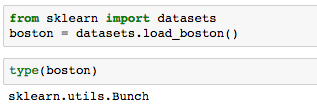The output of the second cell tells us that it's a scikit-learn Bunch object. Let's get some more information about that to understand what we are dealing with.

3. Run the next cell to import the base object from scikit-learn utils and print the docstring in our Notebook: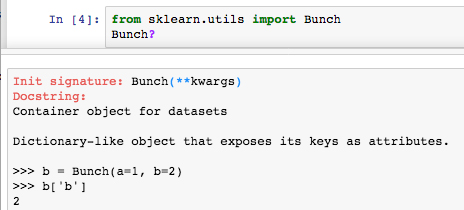Reading the resulting docstring suggests that it's basically a dictionary, and can essentially be treated as such.

4. Print the field names (that is, the keys to the dictionary) by running the next cell.

We find these fields to be self-explanatory: ['DESCR', 'target', 'data', 'feature_names'].

5. Run the next cell to print the dataset description contained in boston['DESCR'].

Note that in this call, we explicitly want to print the field value so that the Notebook renders the content in a more readable format than the string representation (that is, if we just type boston['DESCR'] without wrapping it in a print statement). We then see the dataset information as we've previously summarized:

Boston House Prices dataset
===========================
Notes
------
Data Set Characteristics:
:Number of Instances: 506
:Number of Attributes: 13 numeric/categorical predictive
:Median Value (attribute 14) is usually the target
:Attribute Information (in order):
- CRIM     per capita crime rate by town
â€¦
â€¦
- MEDV     Median value of owner-occupied homes in $1000's :Missing Attribute Values: None Of particular importance here are the feature descriptions (under Attribute Information). We will use this as reference during our analysis. ### Note For the complete code, refer to the Lesson 1.txt file in the Lesson 1 folder. Now, we are going to create a Pandas DataFrame that contains the data. This is beneficial for a few reasons: all of our data will be contained in one object, there are useful and computationally efficient DataFrame methods we can use, and other libraries such as Seaborn have tools that integrate nicely with DataFrames. In this case, we will create our DataFrame with the standard constructor method. 6. Run the cell where Pandas is imported and the docstring is retrieved for pd.DataFrame: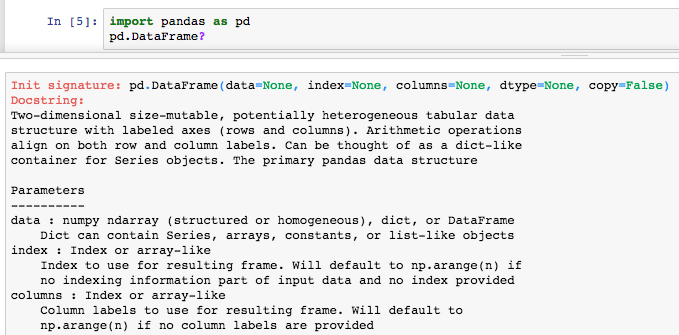The docstring reveals the DataFrame input parameters. We want to feed in boston['data'] for the data and use boston['feature_names'] for the headers. 7. Run the next few cells to print the data, its shape, and the feature names: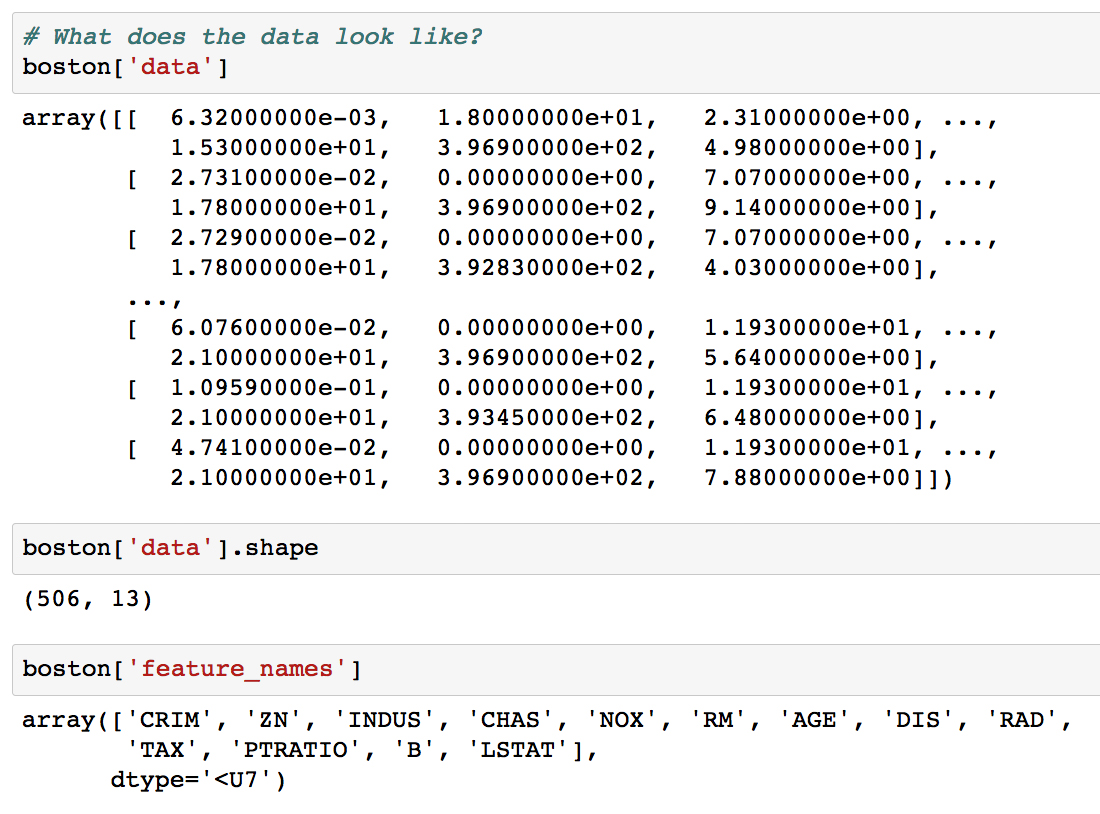Looking at the output, we see that our data is in a 2D NumPy array. Running the command boston['data'].shape returns the length (number of samples) and the number of features as the first and second outputs, respectively. 8. Load the data into a Pandas DataFrame df by running the following: df = pd.DataFrame(data=boston['data'], columns=boston['feature_names']) In machine learning, the variable that is being modeled is called the target variable; it's what you are trying to predict given the features. For this dataset, the suggested target is MEDV, the median house value in 1,000s of dollars. 9. Run the next cell to see the shape of the target: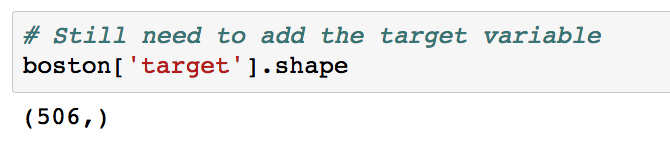We see that it has the same length as the features, which is what we expect. It can therefore be added as a new column to the DataFrame. 10. Add the target variable to df by running the cell with the following: df['MEDV'] = boston['target'] 11. To distinguish the target from our features, it can be helpful to store it at the front of our DataFrame. Move the target variable to the front of df by running the cell with the following: y = df['MEDV'].copy() del df['MEDV'] df = pd.concat((y, df), axis=1) Here, we introduce a dummy variable y to hold a copy of the target column before removing it from the DataFrame. We then use the Pandas concatenation function to combine it with the remaining DataFrame along the 1st axis (as opposed to the 0th axis, which combines rows). ### Note You will often see dot notation used to reference DataFrame columns. For example, previously we could have done y = df.MEDV.copy(). This does not work for deleting columns, however; del df.MEDV would raise an error. 12. Now that the data has been loaded in its entirety, let's take a look at the DataFrame. We can do df.head() or df.tail() to see a glimpse of the data and len(df) to make sure the number of samples is what we expect. Run the next few cells to see the head, tail, and length of df: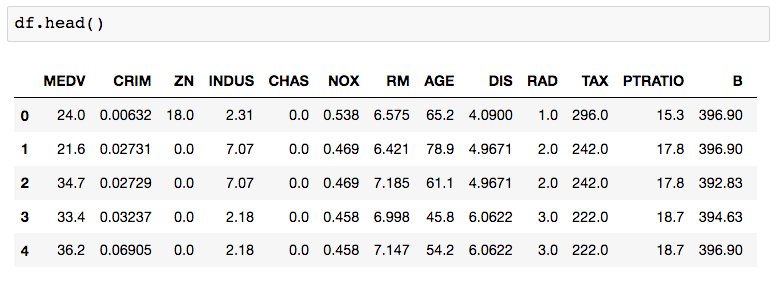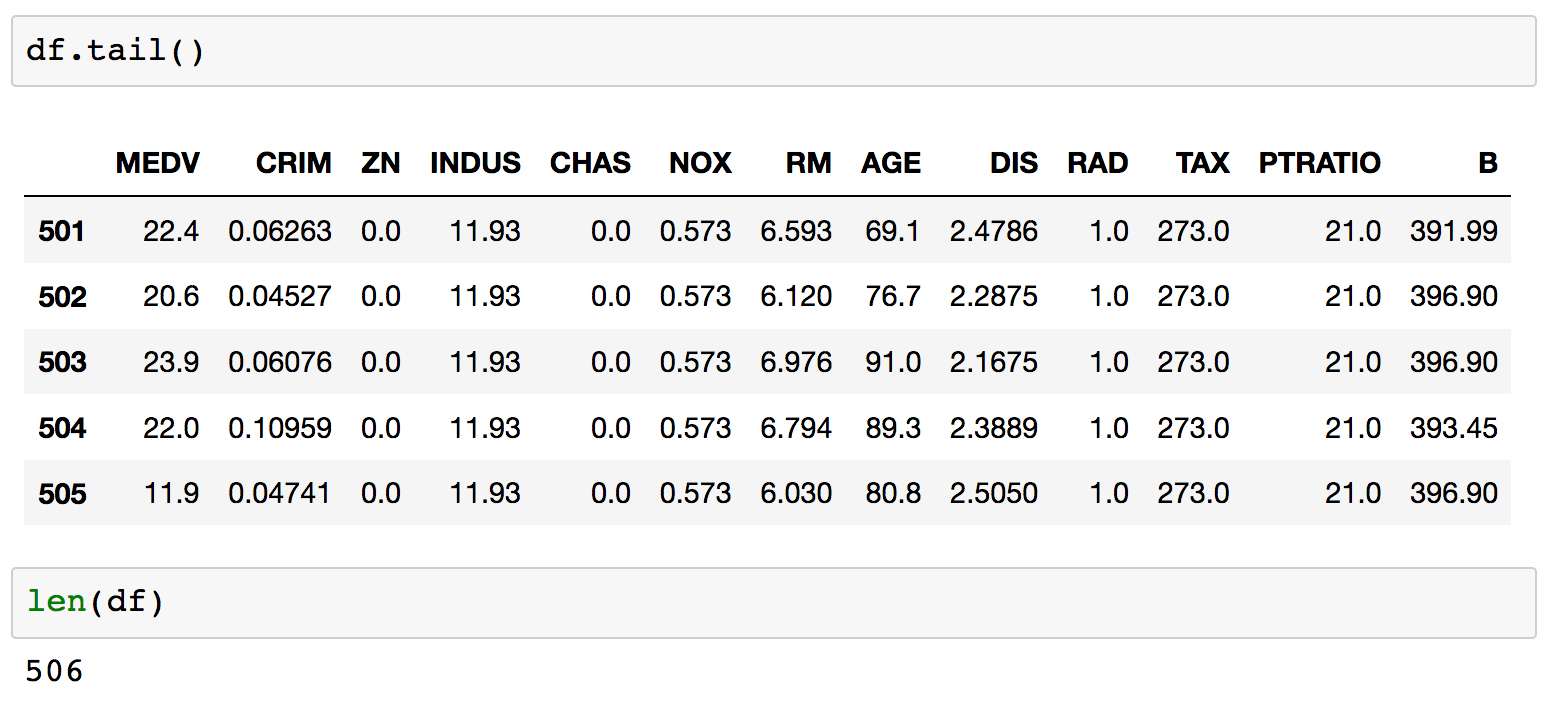Each row is labeled with an index value, as seen in bold on the left side of the table. By default, these are a set of integers starting at 0 and incrementing by one for each row. 13. Printing df.dtypes will show the datatype contained within each column. Run the next cell to see the datatypes of each column. For this dataset, we see that every field is a float and therefore most likely a continuous variable, including the target. This means that predicting the target variable is a regression problem. 14. The next thing we need to do is clean the data by dealing with any missing data, which Pandas automatically sets as NaN values. These can be identified by running df.isnull(), which returns a Boolean DataFrame of the same shape as df. To get the number of NaNs per column, we can do df.isnull().sum(). Run the next cell to calculate the number of NaN values in each column: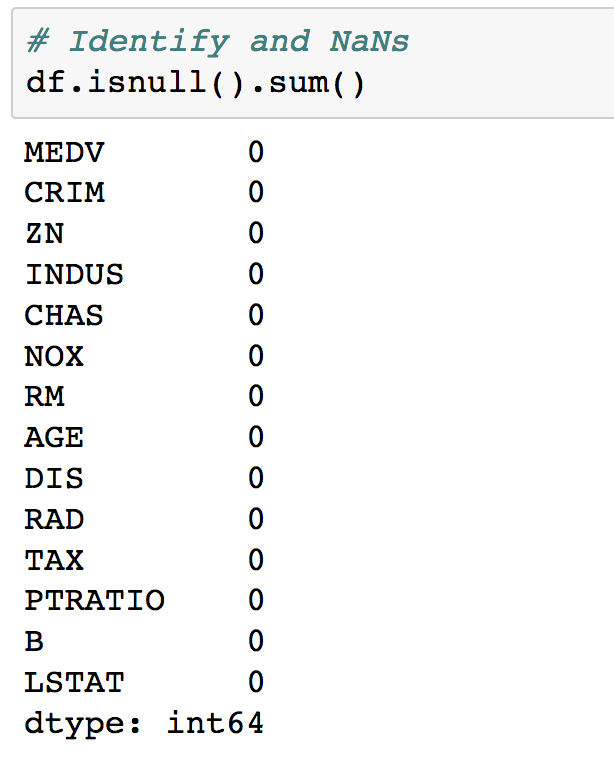For this dataset, we see there are no NaNs, which means we have no immediate work to do in cleaning the data and can move on. 15. To simplify the analysis, the final thing we'll do before exploration is remove some of the columns. We won't bother looking at these, and instead focus on the remainder in more detail. Remove some columns by running the cell that contains the following code: for col in ['ZN', 'NOX', 'RAD', 'PTRATIO', 'B']: del df[col] ### Subtopic B: Data Exploration Since this is an entirely new dataset that we've never seen before, the first goal here is to understand the data. We've already seen the textual description of the data, which is important for qualitative understanding. We'll now compute a quantitative description. #### Explore the Boston housing dataset 1. Navigate to Subtopic B: Data exploration in the Jupyter Notebook and run the cell containing df.describe():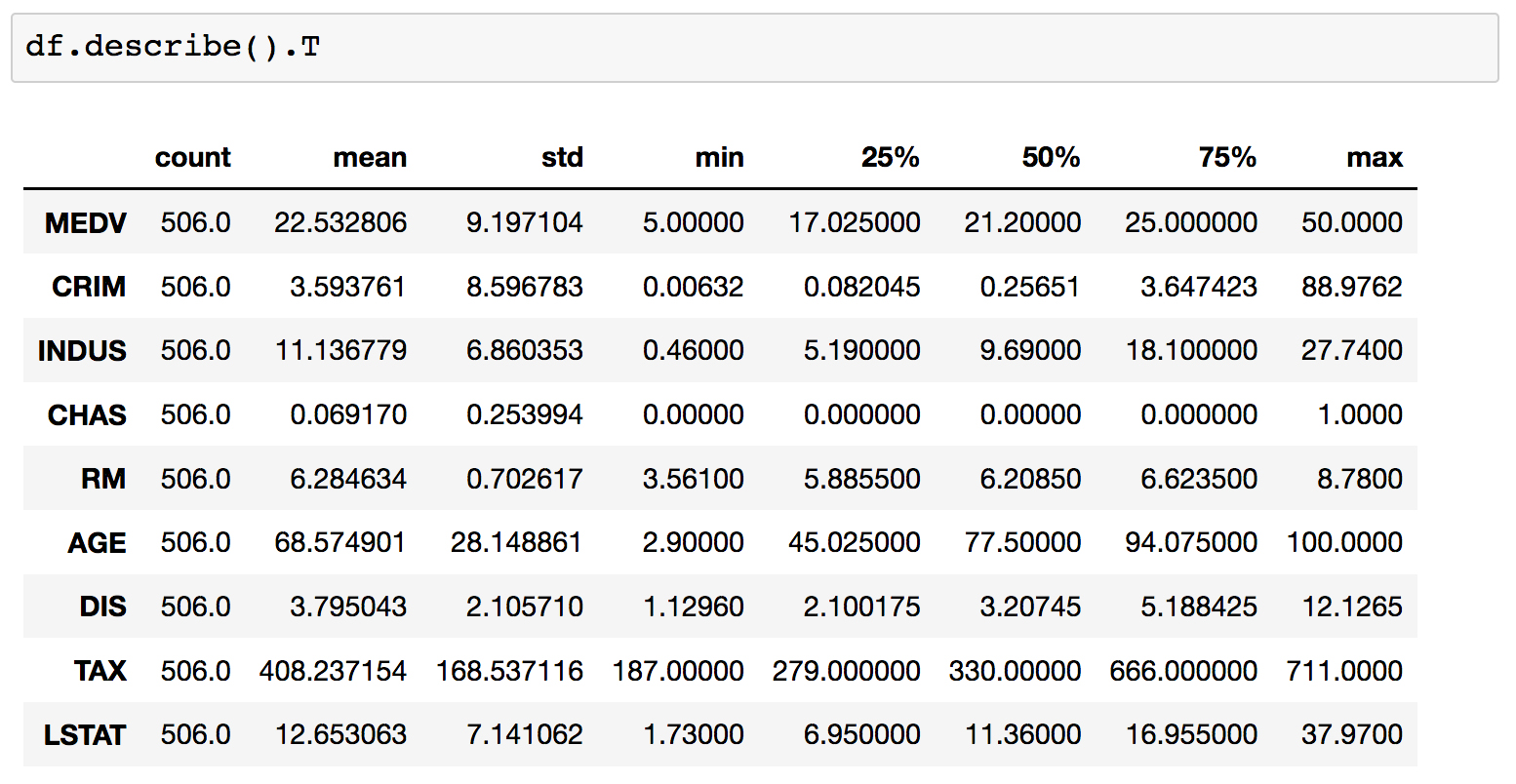This computes various properties including the mean, standard deviation, minimum, and maximum for each column. This table gives a high-level idea of how everything is distributed. Note that we have taken the transform of the result by adding a .T to the output; this swaps the rows and columns. Going forward with the analysis, we will specify a set of columns to focus on. 2. Run the cell where these "focus columns" are defined: cols = ['RM', 'AGE', 'TAX', 'LSTAT', 'MEDV'] 3. This subset of columns can be selected from df using square brackets. Display this subset of the DataFrame by running df[cols].head():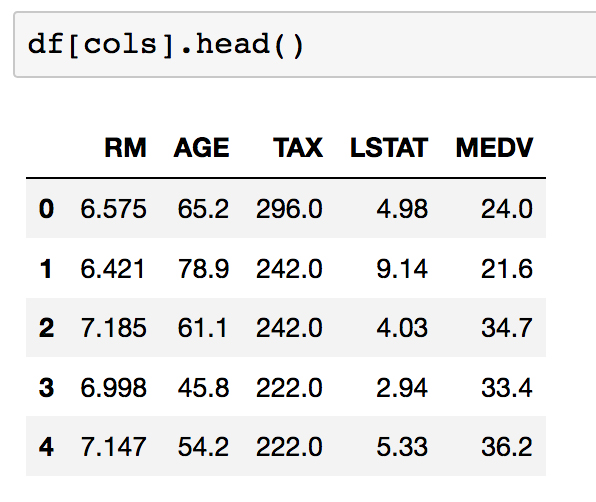As a reminder, let's recall what each of these columns is. From the dataset documentation, we have the following:  - RM average number of rooms per dwelling - AGE proportion of owner-occupied units built prior to 1940 - TAX full-value property-tax rate per$10,000
- LSTAT    % lower status of the population

### Subtopic C: Introduction to Predictive Analytics with Jupyter Notebooks

Continuing our analysis of the Boston housing dataset, we can see that it presents us with a regression problem where we predict a continuous target variable given a set of features. In particular, we'll be predicting the median house value (MEDV). We'll train models that take only one feature as input to make this prediction. This way, the models will be conceptually simple to understand and we can focus more on the technical details of the scikit-learn API. Then, in the next lesson, you'll be more comfortable dealing with the relatively complicated models.

#### Linear models with Seaborn and scikit-learn

1. Scroll to Subtopic C: Introduction to predictive analytics in the Jupyter Notebook and look just above at the pairplot we created in the previous section. In particular, look at the scatter plots in the bottom-left corner: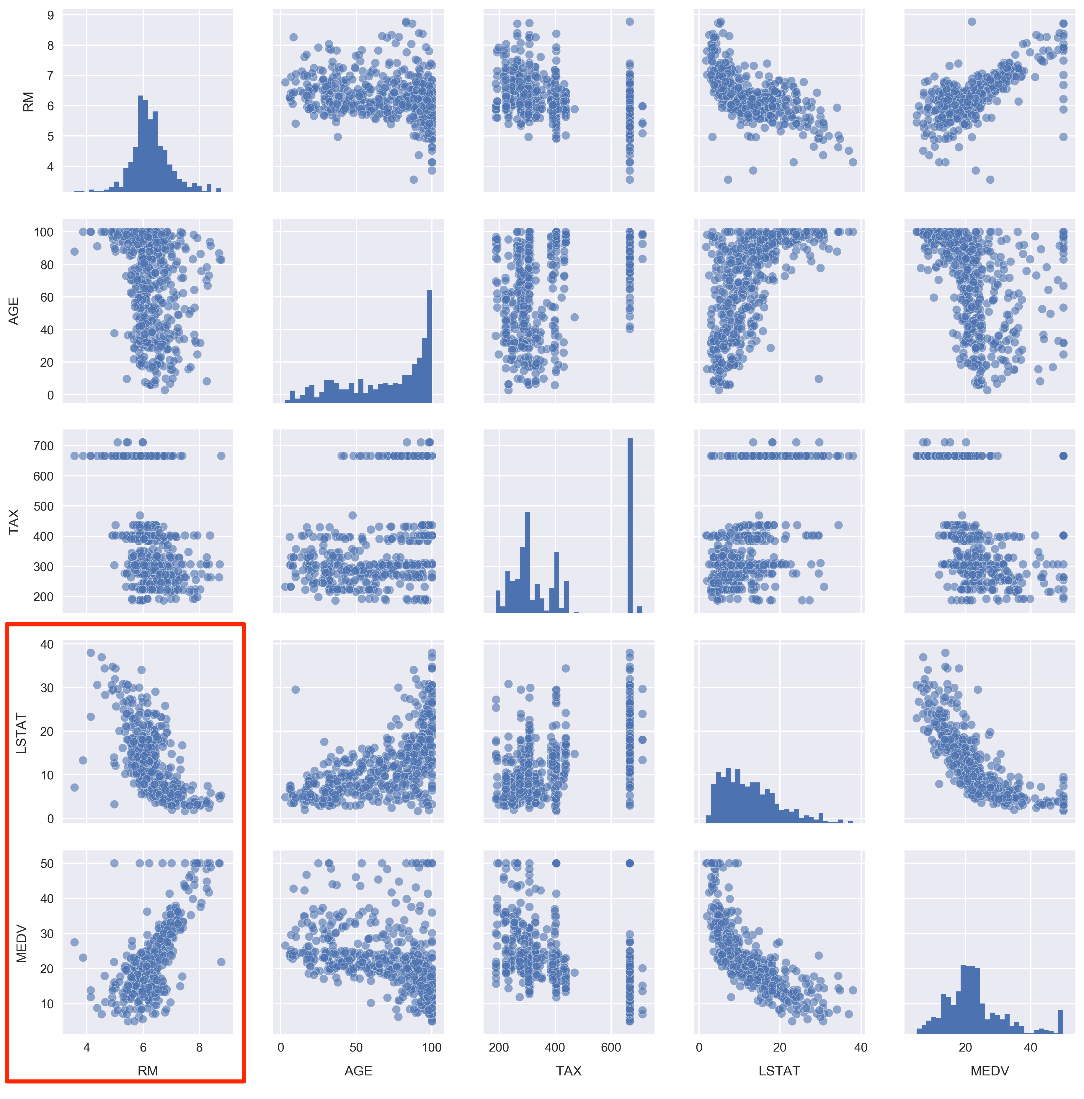Note how the number of rooms per house (RM) and the % of the population that is lower class (LSTAT) are highly correlated with the median house value (MDEV). Let's pose the following question: how well can we predict MDEV given these variables?

To help answer this, let's first visualize the relationships using Seaborn. We will draw the scatter plots along with the line of best fit linear models.

2. Draw scatter plots along with the linear models by running the cell that contains the following:

fig, ax = plt.subplots(1, 2)
sns.regplot('RM', 'MEDV', df, ax=ax,
scatter_kws={'alpha': 0.4}))
sns.regplot('LSTAT', 'MEDV', df, ax=ax,
scatter_kws={'alpha': 0.4}))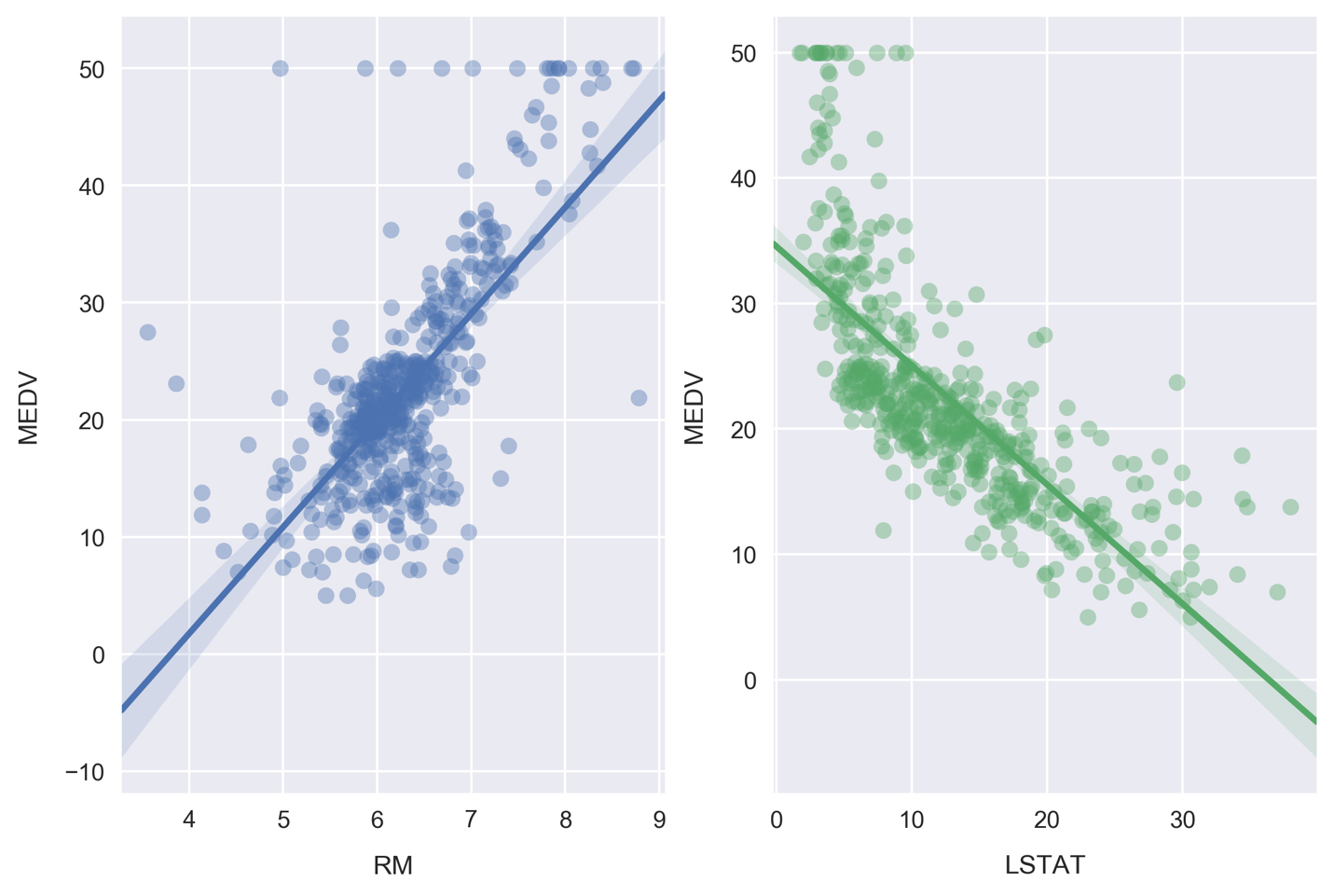The line of best fit is calculated by minimizing the ordinary least squares error function, something Seaborn does automatically when we call the regplot function. Also note the shaded areas around the lines, which represent 95% confidence intervals.

### Note

These 95% confidence intervals are calculated by taking the standard deviation of data in bins perpendicular to the line of best fit, effectively determining the confidence intervals at each point along the line of best fit. In practice, this involves Seaborn bootstrapping the data, a process where new data is created through random sampling with replacement. The number of bootstrapped samples is automatically determined based on the size of the dataset, but can be manually set as well by passing the n_boot argument.

3. Seaborn can also be used to plot the residuals for these relationships. Plot the residuals by running the cell containing the following:

fig, ax = plt.subplots(1, 2)
ax = sns.residplot('RM', 'MEDV', df, ax=ax,
scatter_kws={'alpha': 0.4})
ax.set_ylabel('MDEV residuals $(y-\hat{y})$')
ax = sns.residplot('LSTAT', 'MEDV', df, ax=ax,
scatter_kws={'alpha': 0.4})
ax.set_ylabel('')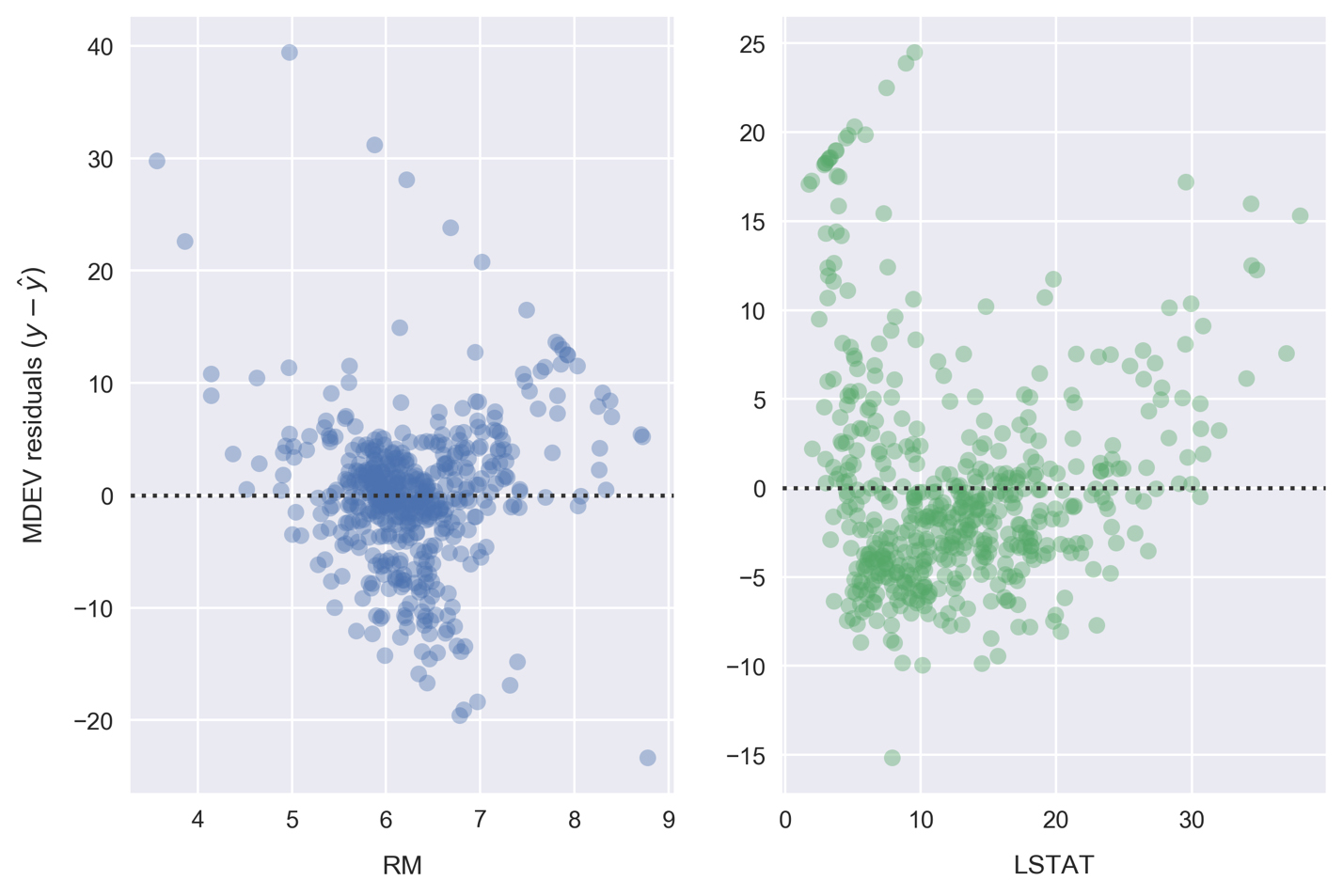Each point on these residual plots is the difference between that sample (y) and the linear model prediction (Å·). Residuals greater than zero are data points that would be underestimated by the model. Likewise, residuals less than zero are data points that would be overestimated by the model.

Patterns in these plots can indicate suboptimal modeling. In each preceding case, we see diagonally arranged scatter points in the positive region. These are caused by the $50,000 cap on MEDV. The RM data is clustered nicely around 0, which indicates a good fit. On the other hand, LSTAT appears to be clustered lower than 0. 4. Moving on from visualizations, the fits can be quantified by calculating the mean squared error. We'll do this now using scikit-learn. Define a function that calculates the line of best fit and mean squared error, by running the cell that contains the following: def get_mse(df, feature, target='MEDV'): # Get x, y to model y = df[target].values x = df[feature].values.reshape(-1,1) ... ... error = mean_squared_error(y, y_pred) print('mse = {:.2f}'.format(error)) print() ### Note For the complete code, refer to the Lesson 1.txt file in the Lesson 1 folder. In the get_mse function, we first assign the variables y and x to the target MDEV and the dependent feature, respectively. These are cast as NumPy arrays by calling the values attribute. The dependent features array is reshaped to the format expected by scikit-learn; this is only necessary when modeling a one-dimensional feature space. The model is then instantiated and fitted on the data. For linear regression, the fitting consists of computing the model parameters using the ordinary least squares method (minimizing the sum of squared errors for each sample). Finally, after determining the parameters, we predict the target variable and use the results to calculate the MSE. 5. Call the get_mse function for both RM and LSTAT, by running the cell containing the following: get_mse(df, 'RM') get_mse(df, 'LSTAT')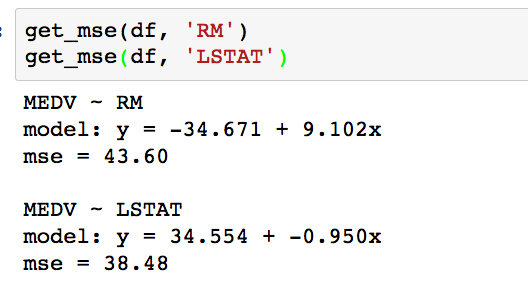Comparing the MSE, it turns out the error is slightly lower for LSTAT. Looking back to the scatter plots, however, it appears that we might have even better success using a polynomial model for LSTAT. In the next activity, we will test this by computing a third-order polynomial model with scikit-learn. Forgetting about our Boston housing dataset for a minute, consider another real-world situation where you might employ polynomial regression. The following example is modeling weather data. In the following plot, we see temperatures (lines) and precipitations (bars) for Vancouver, BC, Canada: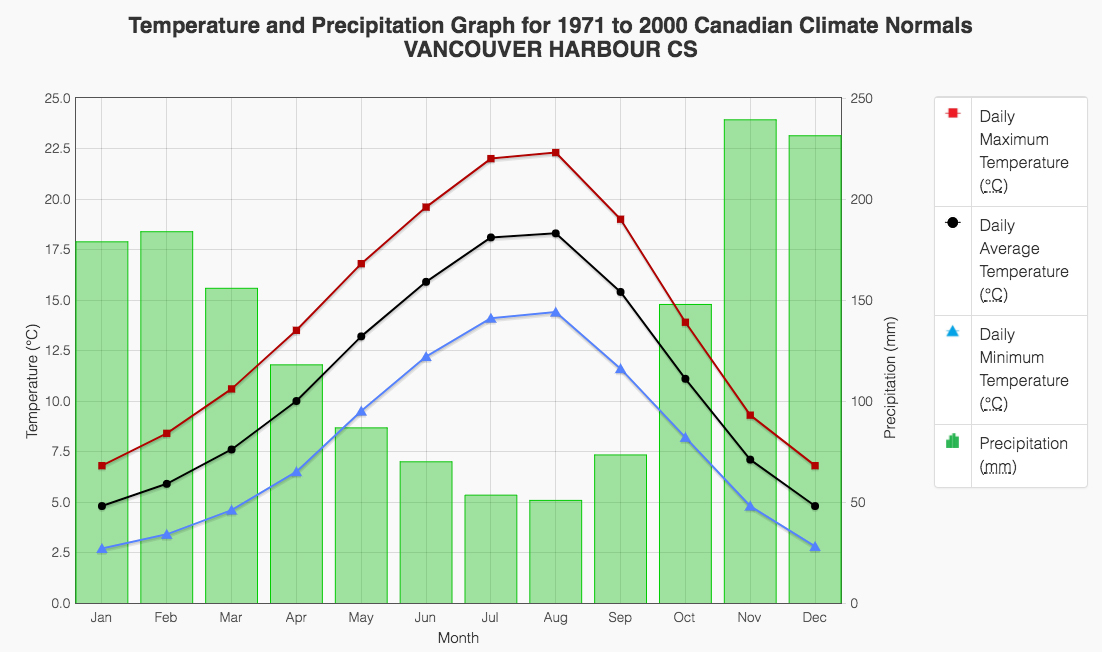Any of these fields are likely to be fit quite well by a fourth-order polynomial. This would be a very valuable model to have, for example, if you were interested in predicting the temperature or precipitation for a continuous range of dates. You can find the data source for this here: http://climate.weather.gc.ca/climate_normals/results_e.html?stnID=888. ### Activity B: Building a Third-Order Polynomial Model Shifting our attention back to the Boston housing dataset, we would like to build a third-order polynomial model to compare against the linear one. Recall the actual problem we are trying to solve: predicting the median house value, given the lower class population percentage. This model could benefit a prospective Boston house purchaser who cares about how much of their community would be lower class. Use scikit-learn to fit a polynomial regression model to predict the median house value (MEDV), given the LSTAT values. We are hoping to build a model that has a lower mean-squared error (MSE). 1. Scroll to the empty cells at the bottom of Subtopic C in your Jupyter Notebook. These will be found beneath the linear-model MSE calculation cell under the Activity heading. ### Note You should fill these empty cells in with code as we complete the activity. You may need to insert new cells as these become filled up; please do so as needed! 2. Given that our data is contained in the DataFrame df, we will first pull out our dependent feature and target variable using the following: y = df['MEDV'].values x = df['LSTAT'].values.reshape(-1,1) This is identical to what we did earlier for the linear model. 3. Check out what x looks like by printing the first few samples with print(x[:3]):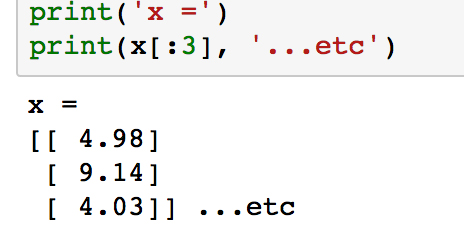Notice how each element in the array is itself an array with length 1. This is what reshape(-1,1) does, and it is the form expected by scikit-learn. 4. Next, we are going to transform x into "polynomial features". The rationale for this may not be immediately obvious but will be explained shortly. Import the appropriate transformation tool from scikit-learn and instantiate the third-degree polynomial feature transformer: from sklearn.preprocessing import PolynomialFeatures poly = PolynomialFeatures(degree=3) 5. At this point, we simply have an instance of our feature transformer. Now, let's use it to transform the LSTAT feature (as stored in the variable x) by running the fit_transform method. Build the polynomial feature set by running the following code: x_poly = poly.fit_transform(x) 6. Check out what x_poly looks like by printing the first few samples with print(x_poly[:3]).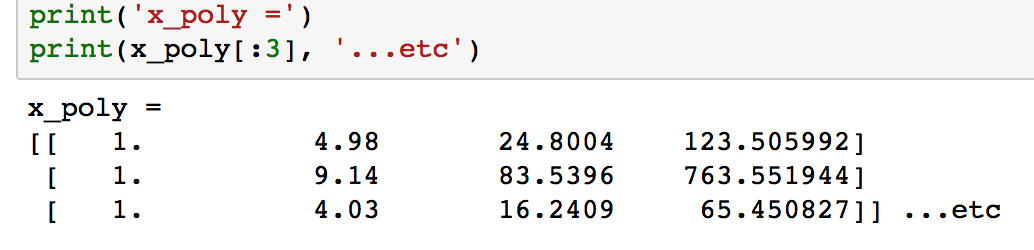Unlike x, the arrays in each row now have length 4, where the values have been calculated as x0, x1, x2 and x3. We are now going to use this data to fit a linear model. Labeling the features as a, b, c, and d, we will calculate the coefficients Î±0, Î±1, Î±2, and Î±3 and of the linear model: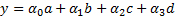We can plug in the definitions of a, b, c, and d, to get the following polynomial model, where the coefficients are the same as the previous ones: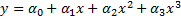7. We'll import the LinearRegression class and build our linear classification model the same way as before, when we calculated the MSE. Run the following: from sklearn.linear_model import LinearRegression clf = LinearRegression() clf.fit(x_poly, y) 8. Extract the coefficients and print the polynomial model using the following code: a_0 = clf.intercept_ + clf.coef_ # intercept a_1, a_2, a_3 = clf.coef_[1:] # other coefficients msg = 'model: y = {:.3f} + {:.3f}x + {:.3f}x^2 + {:.3f}x^3'\ .format(a_0, a_1, a_2, a_3) print(msg)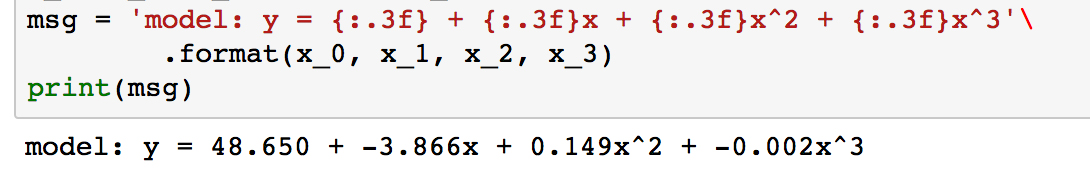To get the actual model intercept, we have to add the intercept_ and coef_ attributes. The higher-order coefficients are then given by the remaining values of coef_. 9. Determine the predicted values for each sample and calculate the residuals by running the following code: y_pred = clf.predict(x_poly) resid_MEDV = y - y_pred 10. Print some of the residual values by running print(resid_MEDV[:10]):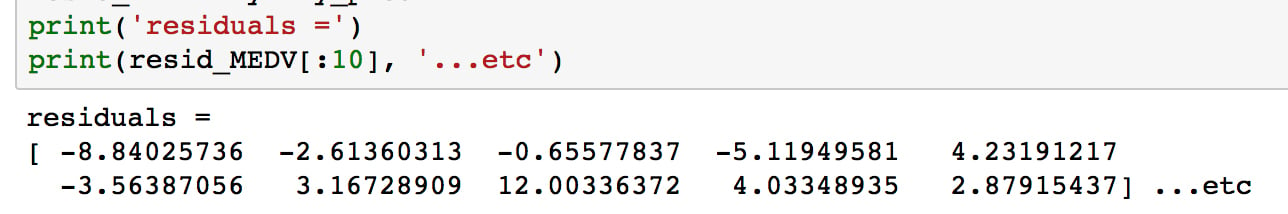We'll plot these soon to compare with the linear model residuals, but first we will calculate the MSE. 11. Run the following code to print the MSE for the third-order polynomial model: from sklearn.metrics import mean_squared_error error = mean_squared_error(y, y_pred) print('mse = {:.2f}'.format(error))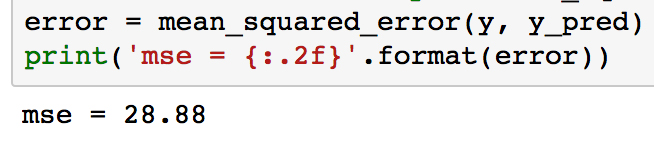As can be seen, the MSE is significantly less for the polynomial model compared to the linear model (which was 38.5). This error metric can be converted to an average error in dollars by taking the square root. Doing this for the polynomial model, we find the average error for the median house value is only$5,300.

Now, we'll visualize the model by plotting the polynomial line of best fit along with the data.

12. Plot the polynomial model along with the samples by running the following:

fig, ax = plt.subplots()
# Plot the samples
ax.scatter(x.flatten(), y, alpha=0.6)
# Plot the polynomial model
x_ = np.linspace(2, 38, 50).reshape(-1, 1)
x_poly = poly.fit_transform(x_)
y_ = clf.predict(x_poly)
ax.plot(x_, y_, color='red', alpha=0.8)
ax.set_xlabel('LSTAT'); ax.set_ylabel('MEDV');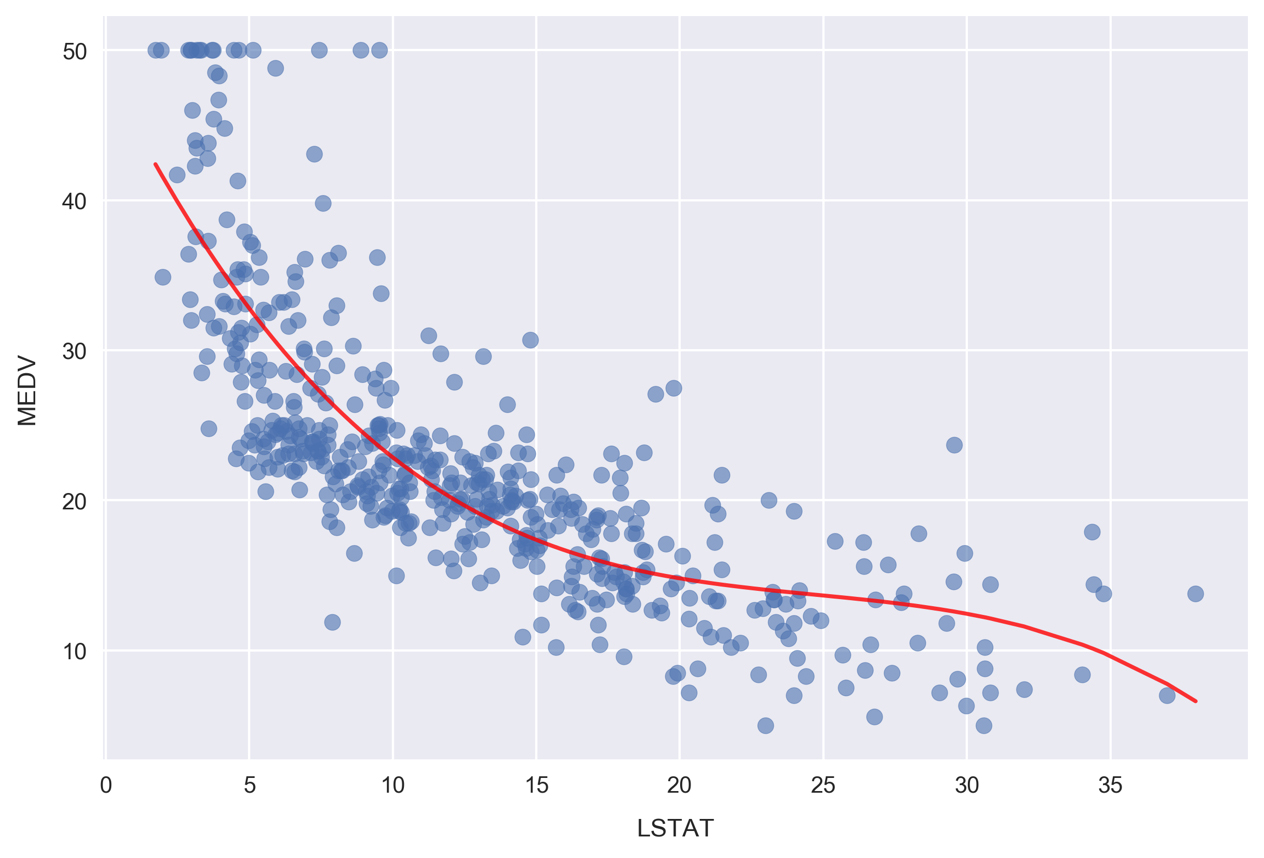Here, we are plotting the red curve by calculating the polynomial model predictions on an array of x values. The array of x values was created using np.linspace, resulting in 50 values arranged evenly between 2 and 38.

Now, we'll plot the corresponding residuals. Whereas we used Seaborn for this earlier, we'll have to do it manually to show results for a scikit-learn model. Since we already calculated the residuals earlier, as reference by the resid_MEDV variable, we simply need to plot this list of values on a scatter chart.

13. Plot the residuals by running the following:

fig, ax = plt.subplots(figsize=(5, 7))
ax.scatter(x, resid_MEDV, alpha=0.6)
ax.set_xlabel('LSTAT')
ax.set_ylabel('MEDV Residual $(y-\hat{y})$')
plt.axhline(0, color='black', ls='dotted');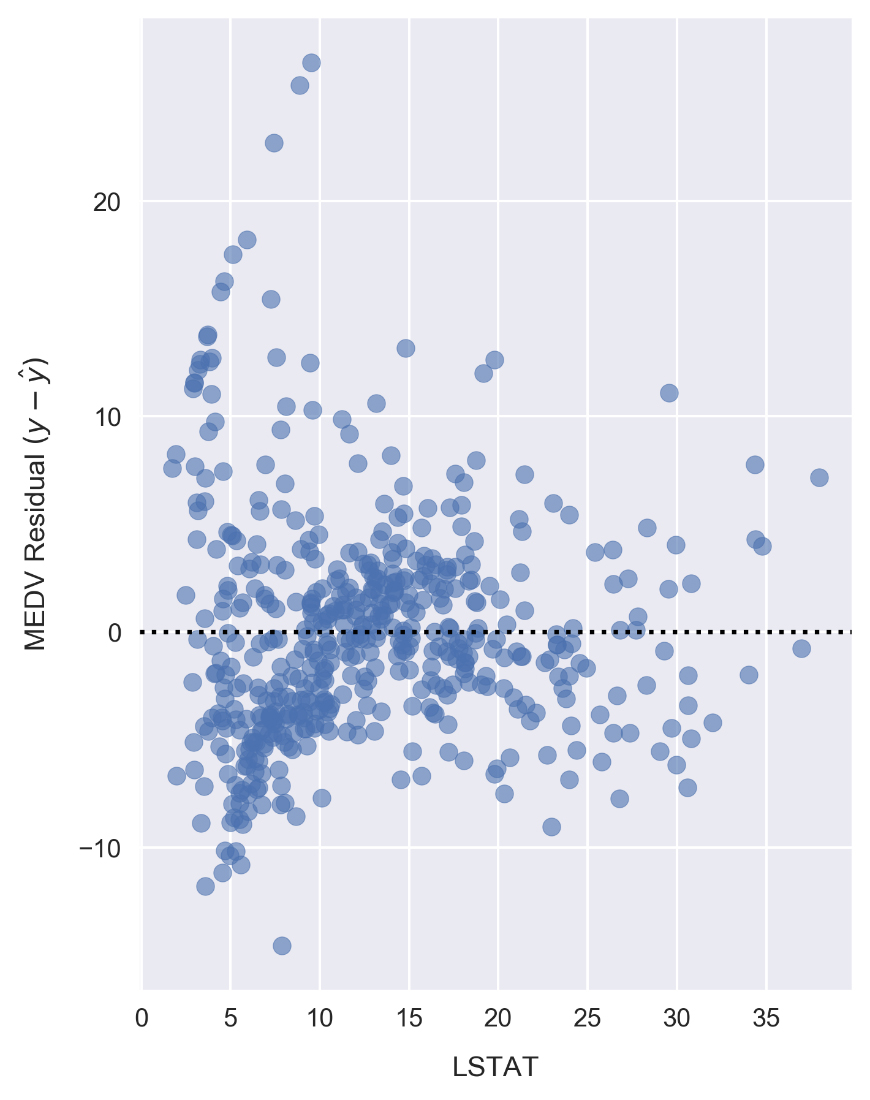Compared to the linear model LSTAT residual plot, the polynomial model residuals appear to be more closely clustered around y - Å· = 0. Note that y is the sample MEDV and Å· is the predicted value. There are still clear patterns, such as the cluster near x = 7 and y = -7 that indicates suboptimal modeling.

Having successfully modeled the data using a polynomial model, let's finish up this lesson by looking at categorical features. In particular, we are going to build a set of categorical features and use them to explore the dataset in more detail.

### Subtopic D: Using Categorical Features for Segmentation Analysis

Often, we find datasets where there are a mix of continuous and categorical fields. In such cases, we can learn about our data and find patterns by segmenting the continuous variables with the categorical fields.

As a specific example, imagine you are evaluating the return on investment from an ad campaign. The data you have access to contain measures of some calculated return on investment (ROI) metric. These values were calculated and recorded daily and you are analyzing data from the previous year. You have been tasked with finding data-driven insights on ways to improve the ad campaign. Looking at the ROI daily timeseries, you see a weekly oscillation in the data. Segmenting by day of the week, you find the following ROI distributions (where 0 represents the first day of the week and 6 represents the last).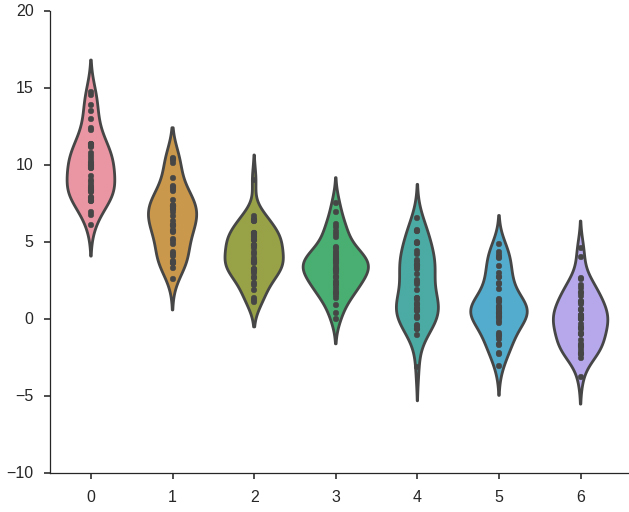This shows clearly that the ad campaign achieves the largest ROI near the beginning of the week, tapering off later. The recommendation, therefore, may be to reduce ad spending in the latter half of the week. To continue searching for insights, you could also imagine repeating the same process for ROI grouped by month.

Since we don't have any categorical fields in the Boston housing dataset we are working with, we'll create one by effectively discretizing a continuous field. In our case, this will involve binning the data into "low", "medium", and "high" categories. It's important to note that we are not simply creating a categorical data field to illustrate the data analysis concepts in this section. As will be seen, doing this can reveal insights from the data that would otherwise be difficult to notice or altogether unavailable.

#### Create categorical fields from continuous variables and make segmented visualizations

1. Scroll up to the pairplot in the Jupyter Notebook where we compared MEDV, LSTAT, TAX, AGE, and RM: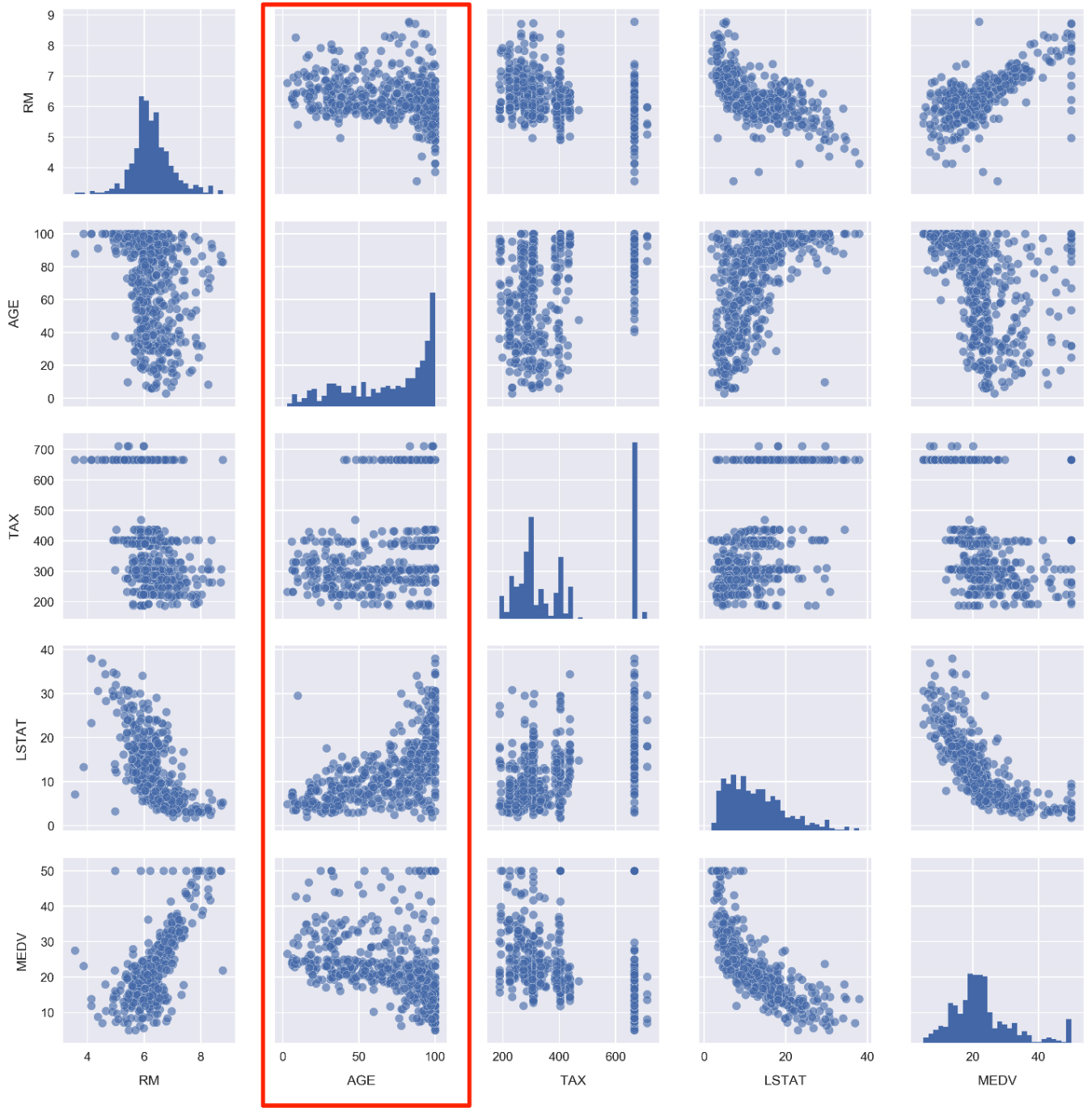Take a look at the panels containing AGE. As a reminder, this feature is defined as the proportion of owner-occupied units built prior to 1940. We are going to convert this feature to a categorical variable. Once it's been converted, we'll be able to replot this figure with each panel segmented by color according to the age category.

2. Scroll down to Subtopic D: Building and exploring categorical features and click into the first cell. Type and execute the following to plot the AGE cumulative distribution:

sns.distplot(df.AGE.values, bins=100,
hist_kws={'cumulative': True},
kde_kws={'lw': 0})
plt.xlabel('AGE')
plt.ylabel('CDF')
plt.axhline(0.33, color='red')
plt.axhline(0.66, color='red')
plt.xlim(0, df.AGE.max());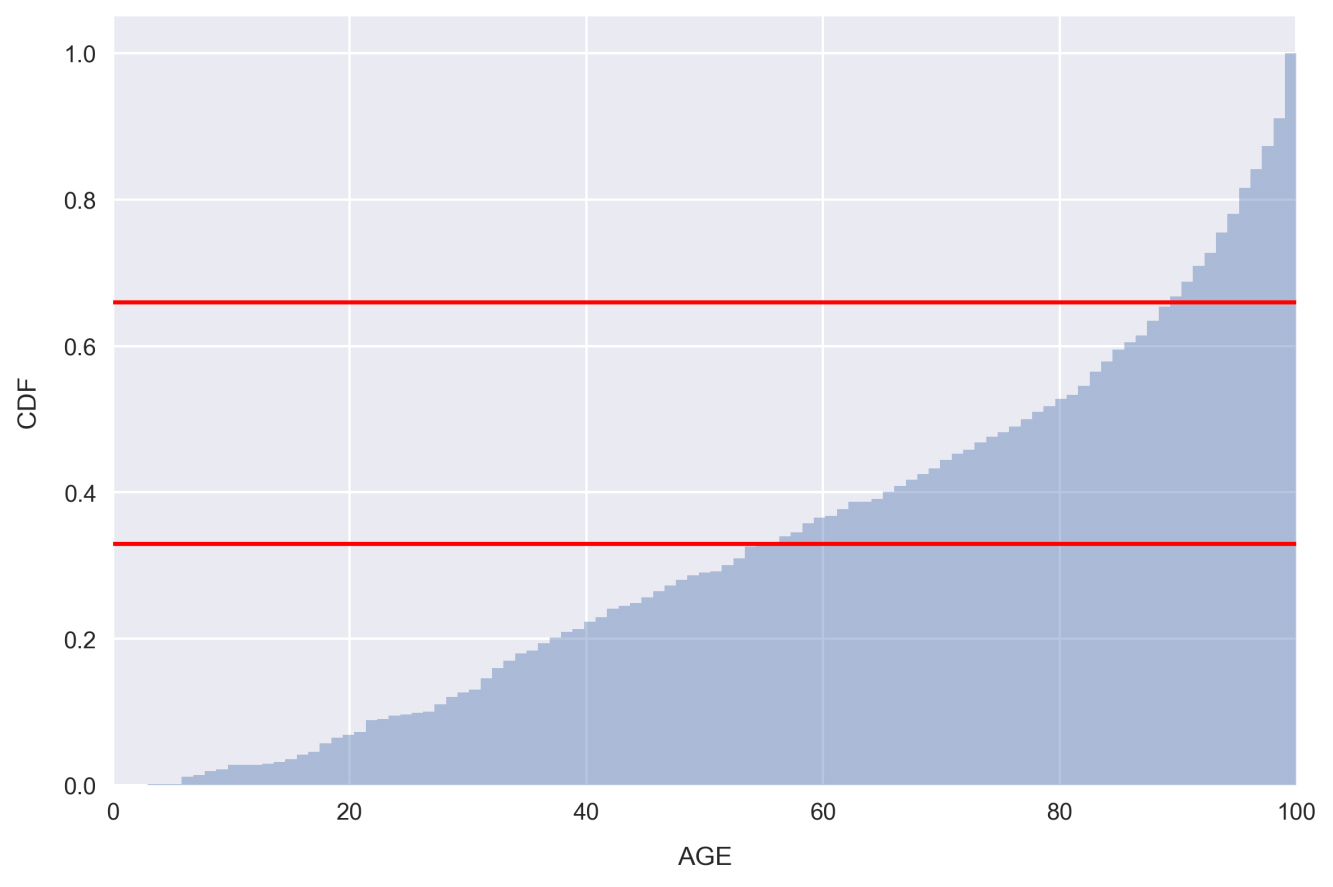Note that we set kde_kws={'lw': 0} in order to bypass plotting the kernel density estimate in the preceding figure.

Looking at the plot, there are very few samples with low AGE, whereas there are far more with a very large AGE. This is indicated by the steepness of the distribution on the far right-hand side.

The red lines indicate 1/3 and 2/3 points in the distribution. Looking at the places where our distribution intercepts these horizontal lines, we can see that only about 33% of the samples have AGE less than 55 and 33% of the samples have AGE greater than 90! In other words, a third of the housing communities have less than 55% of homes built prior to 1940. These would be considered relatively new communities. On the other end of the spectrum, another third of the housing communities have over 90% of homes built prior to 1940. These would be considered very old.

We'll use the places where the red horizontal lines intercept the distribution as a guide to split the feature into categories: Relatively New, Relatively Old, and Very Old.

3. Setting the segmentation points as 50 and 85, create a new categorical feature by running the following code:

def get_age_category(x):
if x < 50:
return 'Relatively New'
elif 50 <= x < 85:
return 'Relatively Old'
else:
return 'Very Old'
df['AGE_category'] = df.AGE.apply(get_age_category)

Here, we are using the very handy Pandas method apply, which applies a function to a given column or set of columns. The function being applied, in this case get_age_category, should take one argument representing a row of data and return one value for the new column. In this case, the row of data being passed is just a single value, the AGE of the sample.

### Note

The apply method is great because it can solve a variety of problems and allows for easily readable code. Often though, vectorized methods such as pd.Series.str can accomplish the same thing much faster. Therefore, it's advised to avoid using it if possible, especially when working with large datasets. We'll see some examples of vectorized methods in the upcoming lessons.

4. Check on how many samples we've grouped into each age category by typing df.groupby('AGE_category').size() into a new cell and running it: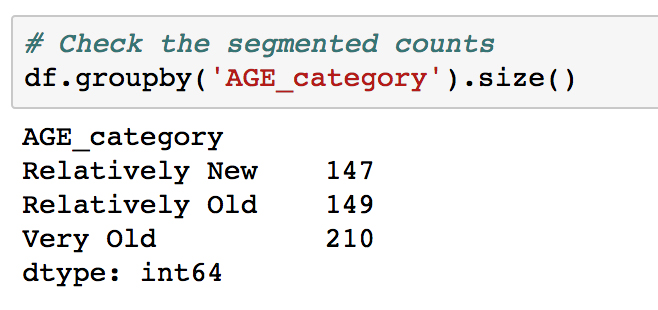Looking at the result, it can be seen that two class sizes are fairly equal, and the Very Old group is about 40% larger. We are interested in keeping the classes comparable in size, so that each is well-represented and it's straightforward to make inferences from the analysis.

### Note

It may not always be possible to assign samples into classes evenly, and in real-world situations, it's very common to find highly imbalanced classes. In such cases, it's important to keep in mind that it will be difficult to make statistically significant claims with respect to the under-represented class. Predictive analytics with imbalanced classes can be particularly difficult. The following blog post offers an excellent summary on methods for handling imbalanced classes when doing machine learning: https://svds.com/learning-imbalanced-classes/.

Let's see how the target variable is distributed when segmented by our new feature AGE_category.

5. Make a violin plot by running the following code:

sns.violinplot(x='MEDV', y='AGE_category', data=df,
order=['Relatively New', 'Relatively Old', 'Very Old']);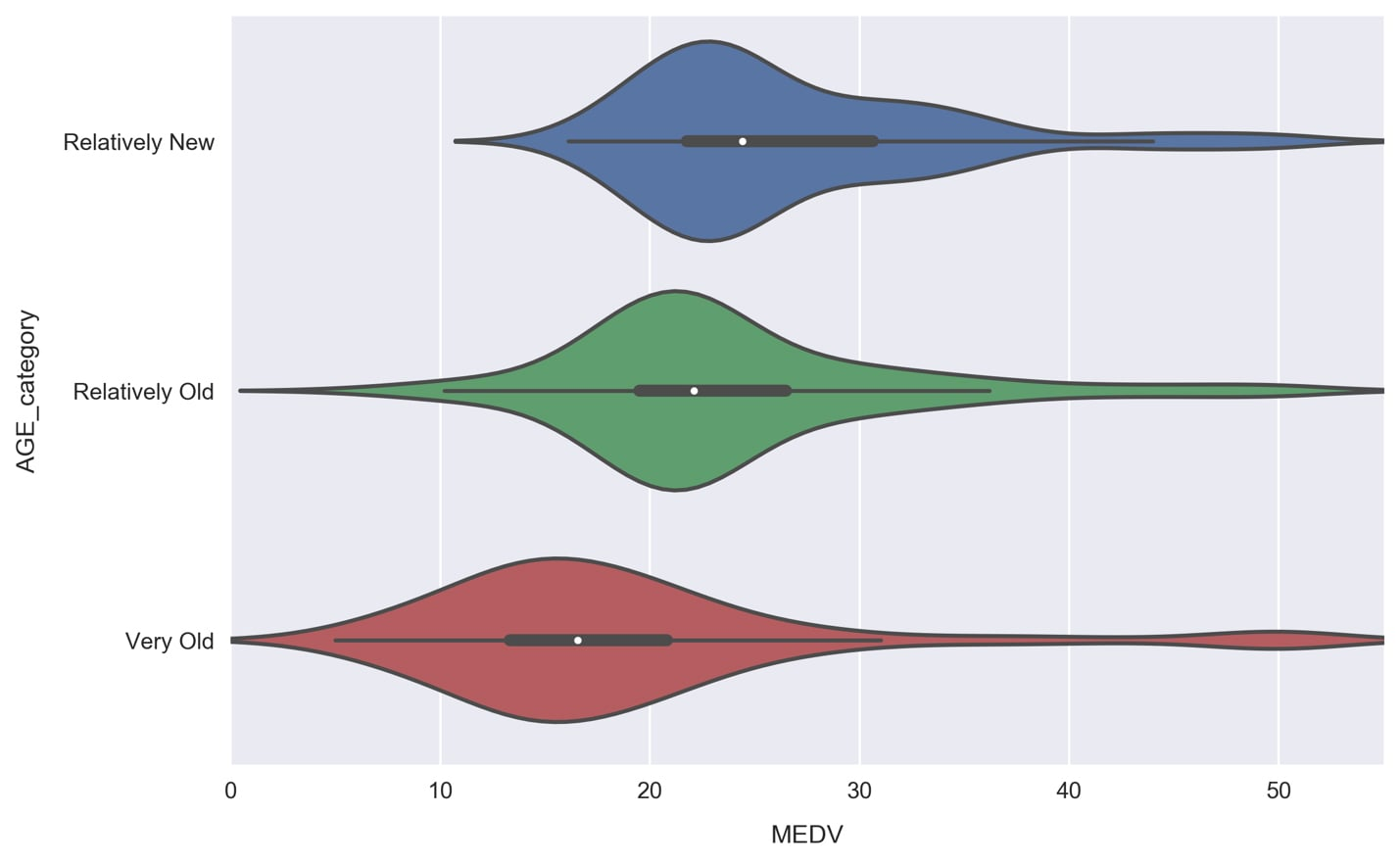The violin plot shows a kernel density estimate of the median house value distribution for each age category. We see that they all resemble a normal distribution. The Very Old group contains the lowest median house value samples and has a relatively large width, whereas the other groups are more tightly centered around their average. The young group is skewed to the high end, which is evident from the enlarged right half and position of the white dot in the thick black line within the body of the distribution.

This white dot represents the mean and the thick black line spans roughly 50% of the population (it fills to the first quantile on either side of the white dot). The thin black line represents boxplot whiskers and spans 95% of the population. This inner visualization can be modified to show the individual data points instead by passing inner='point' to sns.violinplot(). Let's do that now.

6. Redo the violin plot adding the inner='point' argument to the sns.violinplot call: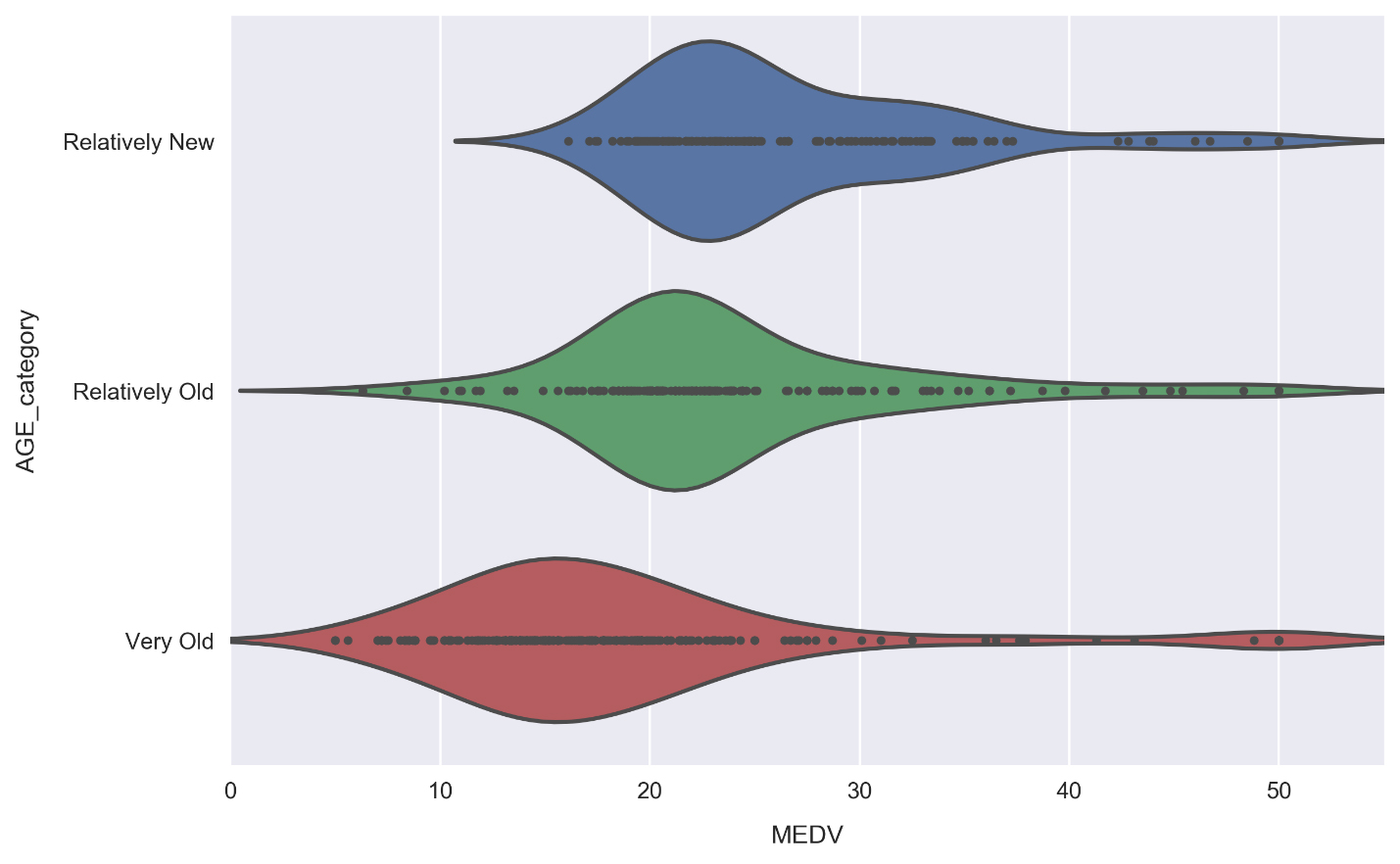It's good to make plots like this for test purposes in order to see how the underlying data connects to the visual. We can see, for example, how there are no median house values lower than roughly \$16,000 for the Relatively New segment, and therefore the distribution tail actually contains no data. Due to the small size of our dataset (only about 500 rows), we can see this is the case for each segment.

7. Re-do the pairplot from earlier, but now include color labels for each AGE category. This is done by simply passing the hue argument, as follows:

cols = ['RM', 'AGE', 'TAX', 'LSTAT', 'MEDV', 'AGE_category']
sns.pairplot(df[cols], hue='AGE_category',
hue_order=['Relatively New', 'Relatively Old', 'Very Old'],
plot_kws={'alpha': 0.5}, diag_kws={'bins': 30});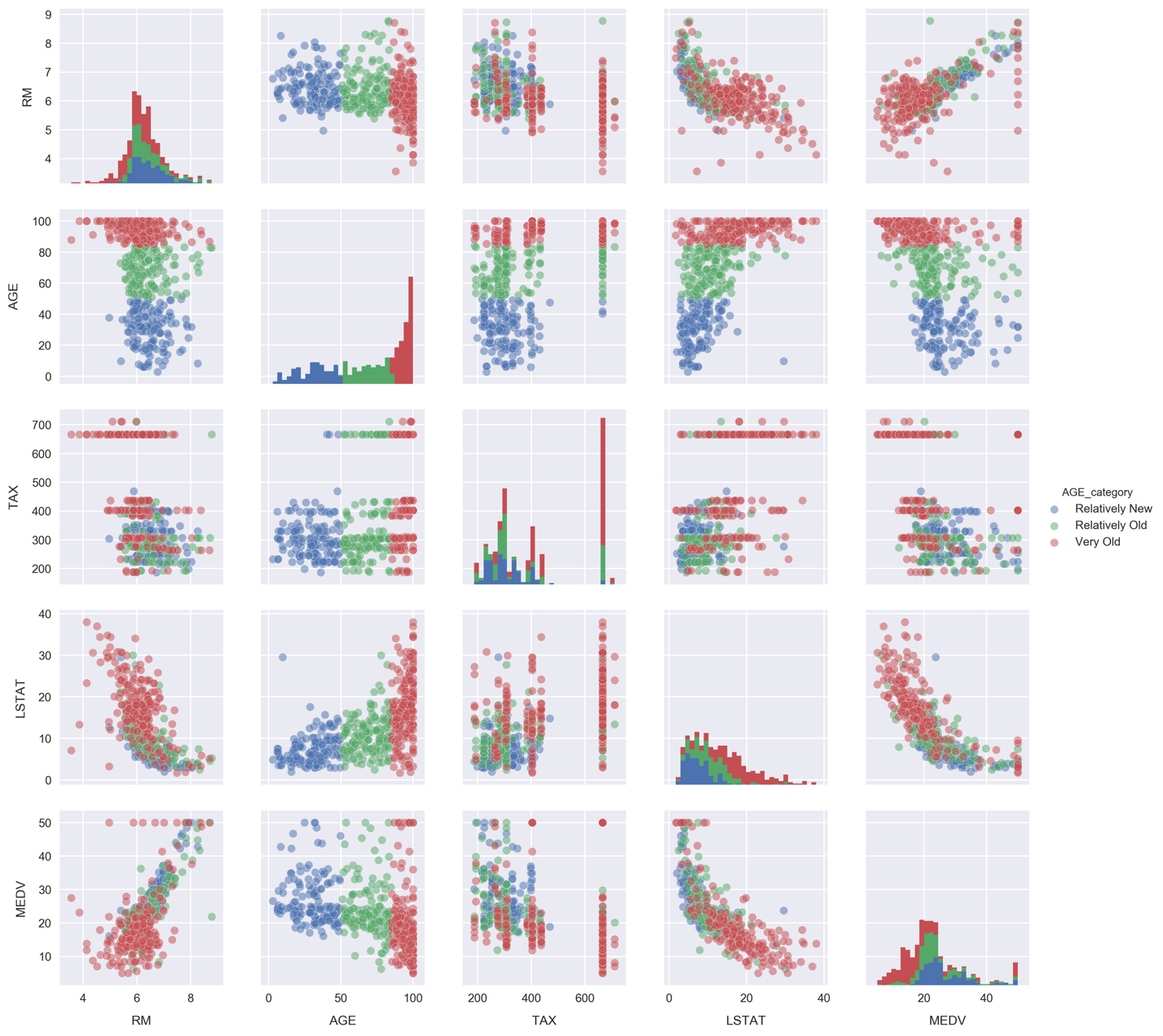Looking at the histograms, the underlying distributions of each segment appear similar for RM and TAX. The LSTAT distributions, on the other hand, look more distinct. We can focus on them in more detail by again using a violin plot.

8. Make a violin plot comparing the LSTAT distributions for each AGE_category segment: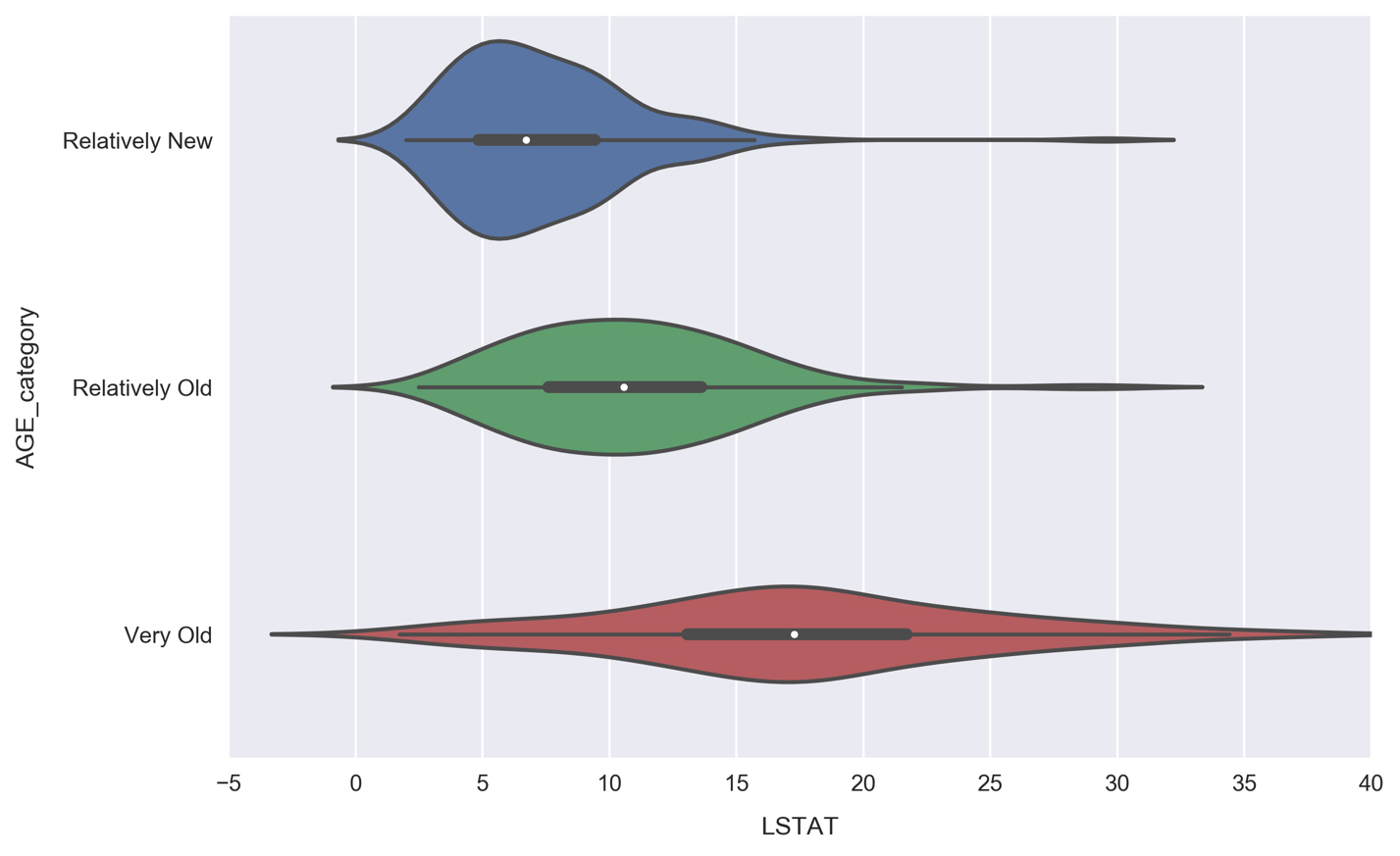Unlike the MEDV violin plot, where each distribution had roughly the same width, here we see the width increasing along with AGE. Communities with primarily old houses (the Very Old segment) contain anywhere from very few to many lower class residents, whereas Relatively New communities are much more likely to be predominantly higher class, with over 95% of samples having less lower class percentages than the Very Old communities. This makes sense, because Relatively New neighborhoods would be more expensive.

## Summary

In this lesson, you have seen the fundamentals of data analysis in Jupyter.

We began with usage instructions and features of Jupyter such as magic functions and tab completion. Then, transitioning to data-science-specific material, we introduced the most important libraries for data science with Python.

In the latter half of the lesson, we ran an exploratory analysis in a live Jupyter Notebook. Here, we used visual assists such as scatter plots, histograms, and violin plots to deepen our understanding of the data. We also performed simple predictive modeling, a topic which will be the focus of the following lesson in this book.

In the next lesson, we will discuss how to approach predictive analytics, what things to consider when preparing the data for modeling, and how to implement and compare a variety of models using Jupyter Notebooks.